# Greek arithmetic, geometry and harmonics: Thales to Plato

Greek arithmetic, geometry and harmonics: Thales to Plato
Greek arithmetic, geometry and harmonics: Thales to Plato Ian Mueller INTRODUCTION: PROCLUS’ HISTORY OF GEOMETRY In a famous passage in Book VII of the Republic starting at Socrates proposes to inquire about the studies (mathēmata) needed to train the young people who will become leaders of the ideal polis he is describing, that is, the subjects that will draw their souls away from the sensible world of becoming to the intelligible world of being and the dialectical study of the Forms. Socrates goes on to discuss five such studies: arithmetic, plane geometry, solid geometry, astronomy and harmonics. The purpose of this chapter is to discuss some important aspects of the development of these mathematical sciences other than astronomy in the Greek world down to the later fourth century. As will become clear, discussion of this topic involves a wide range of interrelated historical, philosophical, and philological questions on many of which opinion still remains sharply divided. My goal here is more to explain what the questions are than to offer answers to them. As a framework for my discussion I shall use a passage from Proclus’ commentary on Book I of Euclid’s Elements ([8.74], 64.7ff.), which is sometimes referred to as the Eudemian summary, on the assumption that its ultimate source is Eudemus’ history of geometry written c.300.1 I shall simply call it Proclus’ history. Proclus begins by mentioning the origin of geometry in Egyptian land-surveying and the origin of arithmetic in Phoenician commercial activity. He then turns to the accomplishments of the Greeks, beginning with the proverbial Thales, standardly supposed to have flourished c.585, of whom he says: Thales, who had traveled to Egypt, was the first to introduce geometry into Greece. He made many discoveries himself and taught his successors the principles for many other discoveries, treating some things in a more universal way, others more in terms of perception. ([8.74], 65.7–11) After a perplexing reference to an otherwise unknown brother of the poet Stesichorus, Proclus turns to Pythagoras, standardly supposed to have been born sometime between c.570 and c.550: Pythagoras transformed the philosophy of geometry into the form of a liberal education, searching in an upward direction for its principles and investigating its theorems immaterially and intellectually. He discovered the doctrine of the irrationals and the construction of the cosmic figures. After him Anaxagoras of Clazomenae applied himself to many questions in geometry, and so did Oinopides of Chios, who was a little younger than Anaxagoras. Both these men are mentioned by Plato in the Lovers [132a5– b3] as having got a reputation in mathematics. Following them, Hippocrates of Chios, who discovered how to square lunes, and Theodoras of Gyrene became eminent in geometry. For Hippocrates wrote a book of elements, the first of those of whom we have any record who did so. ([8.74], 65.7–66.8) At this point Proclus turns to the age of Plato, so that the material just described represents his whole history of sixth- and fifth-century geometry. Anaxagoras is thought to have flourished c.450. Our main source for Theodoras is Plato’s Theaetetus, in which he is represented as an approximate contemporary of Socrates. We should then assign Oinopides and Hippocrates to the last half of the fifth century. I will discuss fifth- and sixth-century mathematical science in the second part of this chapter. As Proclus’ history suggests, we have a clearer idea of specifically scientific work for the late fifth century than we do for the earlier period, for much of which we depend on reports on the quasilegendary Thales and Pythagoras. Proclus’ history is much more detailed for the fourth century. He focuses on Plato and his associates. A natural inference from what he says would be that all mathematical work in the fourth century was ultimately inspired by Plato. I quote only the material from Proclus which mentions the three figures whom I will be discussing in Part One of this chapter: Archytas, Theaetetus and Eudoxus: After these people Plato made a great advance in geometry and the other mathematical sciences because of his concern for them…. At this time… Archytas of Tarentum and Theaetetus of Athens also lived; these people increased the number of theorems and gave them a more scientific organization… Eudoxus of Cnidus, who was…one of those in Plato’s circle, was the first to increase the number of general theorems; he added another three proportionals to the three [already in use]; and, applying the method of analysis, he increased the number of propositions concerning the section, which Plato had first investigated. ([8.74], 66.8–67.8) The last associate of Plato mentioned by Proclus is a Philip of Mende, standardly assumed to be Philip of Opus.2 Proclus concludes his history when he introduces Euclid: Those who have written histories bring the development of the science up to this point. Euclid is not much younger than these people; he brought the elements together, and he gave an order to many propositions of Eudoxus and perfected many of Theaetetus’s; moreover, he gave irrefutable proofs to propositions which had been demonstrated rather loosely by his predecessors. ([8.74], 66.8–68.10) The chronology of Archytas, Theaetetus and Eudoxus is very obscure, but a certain consensus has emerged, based importantly on assumptions about the relationships among their mathematical achievements. Theaetetus is thought to have died in 369 before he was fifty. Eudoxus is said to have lived 53 years; his death year is now generally put around the time of Plato’s (348–7) or shortly thereafter. Archytas is thought to be an approximate contemporary of Plato, and so born in the 420s. The important issue is not the exact dates, but the assumed intellectual ordering: Archytas, Theaetetus, Eudoxus. PART ONE: THE FOURTH CENTURY (1) The Contents of Euclid’s Elements The oldest Greek scientific text relevant to arithmetic, geometry, and solid geometry is Euclid’s Elements. I give a brief description of its contents. Although the proofs of Books I and II make use of the possibility of drawing a circle with a given radius, the propositions are all concerned with straight lines and rectilineal angles and figures. The focus of Book III is the circle and its properties, and in Book IV Euclid treats rectilineal figures inscribed in or circumscribed about circles. In Books I–IV no use is made of the concept of proportionality (x:y :: z:w) and in consequence none—or virtually none—is made of similarity. It seems clear that Euclid chose to postpone the introduction of proportion, even at the cost of making proofs more complicated than they need to have been. Indeed, he sometimes proves essentially equivalent propositions, first independently of the concept of proportion and then —after he has introduced the concept—using it. Moreover, sometimes the proportion-free proof looks like a reworked version of the proof using proportion.3 Book V is a logical tour de force in which Euclid gives a highly abstract definition of proportionality for what he calls magnitudes (megethē) and represents by straight lines. The essential content of the definition may be paraphrased as follows: V, def. 5. x:y :: z:w if and only if whenever a multiple m•x is greater than (equal to or less than) a multiple n•y, m•z is greater than (equal to or less than) n•w, and (V, def. 7) x:y > z:w if for some m and n m•x > n•y and m•z ≼ n•w. Euclid proceeds to prove a number of important laws of proportionality, e.g., alternation (V.16: if x:y :: z:w, then x:z :: y:w) using a strictly formal reduction to the definition and to basic properties of multiplication and size comparison. In Book VI Euclid applies these laws to plane geometric objects. Geometry disappears at the end of Book VI when Euclid turns to arithmetic, the subject of VII–IX. Logically these three books are completely independent of the first six. In them Euclid uses a notion of proportionality specific to numbers, i.e., positive integers, and proves for numbers laws of proportionality already proven for magnitudes in Book V. In Book X there is a kind of unification of arithmetic and geometry. Euclid distinguishes between commensurable and incommensurable magnitudes, again represented by straight lines, and proves: X.5–8. Two magnitudes are commensurable if and only if they have the ratio of a number to a number. I shall briefly discuss the proof of these propositions at the end of section 5, but in the immediate discussion I shall treat this equivalence as something which can be taken for granted as well known to Greek mathematicians of the fourth and perhaps even fifth century. The bulk of Book X is given over to an elaborate classification of certain ‘irrational’ (alogos) straight lines.4 Euclid proves the ‘irrationality’ of a number of straight lines, the most important being: the medial, the side of a square equal to a rectangle with incommensurable ‘rational’ sides; the binomial, the sum of two incommensurable ‘rational’ straight lines; the apotome, the difference of two incommensurable ‘rational’ straight lines. Book XI covers a great deal of elementary solid geometry quite rapidly; by contrast with his procedure in Books I—IV and VI, Euclid appears willing to use proportionality whenever he thinks it simplifies his argumentation. Book XII is characterized by the method used in establishing its principal results, the socalled method of exhaustion. The method of exhaustion is, in fact, a rigorous technique of indefinitely closer approximations to a given magnitude. It depends on what is traditionally called the axiom of Archimedes or Archimedean condition. Euclid purports to prove a form of the ‘axiom’ in: X.1. Two unequal magnitudes being set out, if from the greater there be subtracted a magnitude greater than its half, and from that which is left a magnitude greater than its half, and if this process be repeated continually, there will be left some magnitude which will be less than the lesser magnitude set out. Most of the results proved in Book XII concern solids, but the first and simplest, XII.2, establishes that circles C, C′ are to one another as the squares sq(d) and sq (d′) on their diameters d and d′ (XII.2). To prove this Euclid first shows (XII. 1) that if P and P′ are similar polygons inscribed in C and C′, then P:P′ :: sq(d):sq(d ′). He then argues indirectly by assuming that C is not to C′ as sq(d) is to sq(d′), but that for some plane figure C* which is, say, smaller than C′, C:C* :: sq(d):sq (d′). He inscribes in C′ successively larger polygons P1′, P2′,… (see Figure 8.1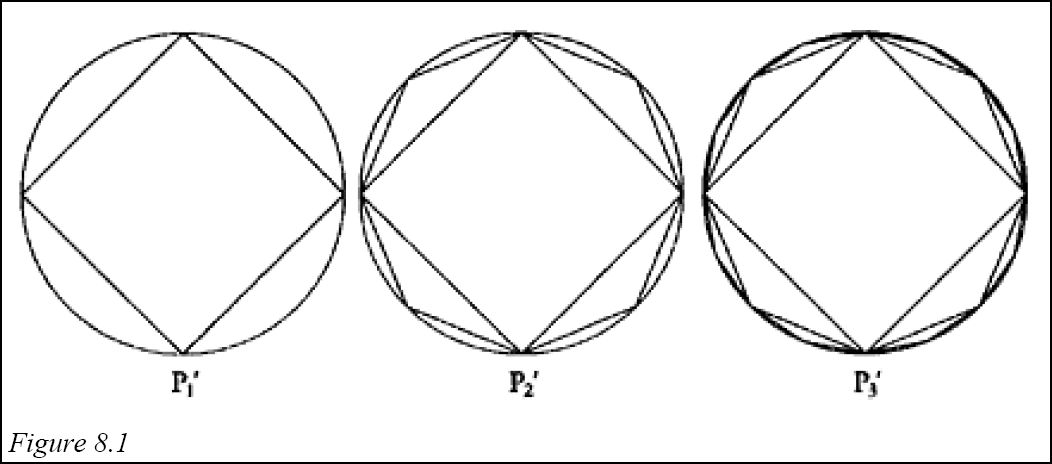) until a Pn′ of greater area than C* is reached, and then inscribes a similar polygon Pn in C. By XII.1, Pn:Pn′ :: sq(d):sq(d1), so that C:C* :: Pn:Pn′, and C:Pn :: C*:Pn′. But this is impossible since C is greater than Pn and C* is less than Pn′. In book XIII Euclid shows in XIII.13–17 how to construct each of the five ‘cosmic figures’ or regular solids, the triangular pyramid contained by four equilateral triangles, the octahedron contained by eight such triangles, the icosahedron contained by twenty, the cube contained by six squares, and the dodecahedron contained by twelve regular pentagons. In XIII. 13–17 Euclid also characterizes the relationship between the edge e of the solid and the diameter d of the circumscribed sphere. For triangular pyramid, cube, and octahedron the results are simply stated; for example, for the triangular pyramid the square on d is 1½ times the square on e. However for the other two solids Euclid uses materials from Book X, taking the diameter d to be a ‘rational’ straight line, and showing that the edge of the dodecahedron is an apotome, and that the icosahedron is a line which he calls minor and defines in X.76. These characterizations of the edges of the regular solids apparently provide the main motivation for the elaborate classification undertaken in Book X. (2) Eudoxus and Books V and XII of Euclid’s Elements Two scholia associate Book V with Eudoxus. The more interesting one says: This book is said to belong to Eudoxus of Cnidus, the mathematician who lived at the time of Plato, but it is nevertheless ascribed to Euclid and not wrongly. For why shouldn’t a thing be assigned to one person as far as its discovery is concerned, even though it is agreed by everyone that it is Euclid’s as far as the arrangement of things with respect to elementhood and with respect to the relations of implication with other things of what has been arranged? ([8.30] 5:282.13–20) It is difficult to make any precise determination of the roles assigned to Eudoxus and Euclid by this scholium, but it would seem that at least some equivalent of the Book V definition of proportionality and an indication of its viability should be ascribed to Eudoxus, and some non-trivial reorganization to Euclid. Book XII is also closely connected with Eudoxus. For in the preface to On Sphere and Cylinder I ([8.22] 1:4.2–13) Archimedes ascribes to him proofs of equivalents of two propositions from Book XII, and in the prefatory letter to The Method ([8.22] 2:430.1–9) he contrasts Eudoxus being the first person to prove these propositions with Democritus being the first to assert one or both of them without proof5. Moreover, in the preface to the Metrica Heron ([8.45] 3:2.13– 18) credits Eudoxus with the first proofs of one of these propositions and of XII. 2. In the prefatory letter to Quadrature of the Parabola ([8.22] 2:264.5–25) Archimedes connects the proof of the equivalents of several propositions in Book XII with what he there calls a lemma and is more or less equivalent to X.1. It seems clear that Eudoxus was responsible for some considerable part of the contents of Book XII, although again we have no way of knowing how much Euclid contributed to its formulation. Since the treatment of proportion in Book V and the use of exhaustion in Book XII can be said to represent the outstanding logical and conceptual achievements of the Elements, there is good reason to compare Eudoxus’ accomplishments with foundational work of the nineteenth century by people such as Weierstrass, Cantor, Dedekind, and Frege.6 (3) Theaetetus and Book XIII of the Elements The Suda ([8.87] 2:689.6–8) says that a Theaetetus of Athens ‘was the first to describe the so-called five solids.’ The connection of Theaetetus with the regular solids is confirmed by a scholion on Book XIII: In this book, the thirteenth, the five figures called Platonic are described. However, they are not Plato’s. Three of the five are Pythagorean, the cube, the pyramid, and the dodecahedron; the octahedron and the icosahedron belong to Theaetetus. They are called Platonic because Plato mentions them in the Timaeus. Euclid put his name also on this book because he extended the elemental ordering to this element as well. ([8.30] 5:654.1–10) We have seen in the introduction that Proclus credits Pythagoras with discovering the construction of the five solids, but his statement is unlikely to be reliable. The most plausible understanding of ancient testimonia about the regular solids has been provided by Waterhouse [8.10], and it confirms both the Suda claim that Theaetetus was the first to describe the five solids and the scholiast’s distinction between the solids of the Pythagoreans and those of Theaetetus. Waterhouse suggests that there was an early recognition of the cube, pyramid, and dodecahedron,7 but that Theaetetus tried to produce a completely general account of the regular solids and brought into geometry both the simple octahedron and the complex icosahedron. As we have seen in section 1, the characterization of the edges of the dodecahedron and icosahedron in Book XIII seems to provide the whole rationale for Book X. And we find Theaetetus connected with Book X as well. (4) Theaetetus and Book X of the Elements: the Three Means and Harmonics In his commentary on Book X of the Elements Pappus of Alexandria describes a relationship between Theaetetus and Book X analogous to the relation between Eudoxus and Book V described by the scholium quoted above: It was Theaetetus who distinguished the powers which are commensurable in length from those which are incommensurable, and who divided the more generally known irrational lines according to the different means, assigning the medial line to geometry, the binomial to arithmetic, and the apotome to harmony, as is stated by Eudemus the Peripatetic. Euclid’s object on the other hand was the attainment of irrefragable principles, which he established for commensurability and incommensurability in general. For rationals and irrationals he formulated definitions and differentiae, determined also many orders of the irrationals, and brought to light whatever of definiteness is to be found in them. ([8.60], I.1) Later Pappus writes: Those who have written concerning these things declare that the Athenian Theaetetus assumed two lines commensurable in square [only] and proved that if he took between them a line in ratio according to geometric proportion, then the line named the medial was produced, but that if he took the line according to arithmetic proportion, then the binomial was produced, and if he took the line according to harmonic proportion, then the apotome was produced. ([8.60], II.17) These assertions require some explication. I begin with the notions of geometric, arithmetic and harmonic proportion, and with a fragment of Archytas’ On Music. There are three musical means, the first arithmetic, the second geometric, the third subcontrary (hupenantios), which is also called harmonic. There is an arithmetic mean when there are three terms in proportion with respect to the same excess: the second term exceeds the third term by as much as the first does the second…. There is a geometric mean when the second term is to the third as the first is to the second… There is a subcontrary mean (which we call harmonic) when the first term exceeds the second by the same part of the first as the middle exceeds the third by a part of the third. (Porphyry [8.73], 93.6–15, DK 47 B 2) Here Archytas speaks of three types of means rather than three types of proportions, although the vocabulary of proportions also slips into what he says. In the present context I shall speak of the three types of mean and use the word ‘proportion’ only for expressions of the form ‘x:y :: z:w’. The geometric mean is, of course, the middle of three terms standing in a standard proportion. The arithmetic mean is simply the arithmetic average of two terms x and z, that is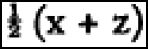. The harmonic mean is usually given a more general definition which we find in Nicomachus ([8.55], II.25.1; cf. Theon of Smyrna [8.92], 114.14–17), according to which: y is the harmonic mean between x and z if and only if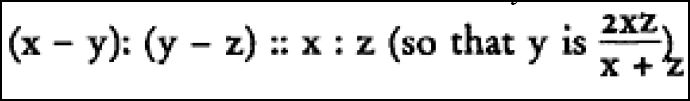It is generally believed that the arithmetic and harmonic means were introduced in connection with harmonics, and with the realization that the fundamental concords of Greek music are expressible by elementary ratios: fourth (doh-fa) 4:3 fifth (doh-sol or fa-doh′) 3:2 octave (doh-doh′) 2:1 The simplest representation of all three of these relations together is: doh fa sol doh′ 12 9 8 6 where 9 is the arithmetic and 8 the harmonic mean between 12 and 6. I shall consider the discovery of these relations in section 2 of Part Two. The important point for now is that harmonics forms part of the background of Theaetetus’s handling of the three ‘generally known’ irrationals.8 The Greek word standing behind ‘power’ in the translation from Pappus is dunamis, which Euclid uses only in the dative: straight lines are said to be commensurable dunamei (in square) when the squares with them as sides are commensurable.9 Pappus’ vocabulary presumably reflects the passage at the beginning of Plato’s Theaetetus (147d–148b) to which he refers. In the passage Theaetetus says that Theodorus was teaching something about dunameis, showing that the three-foot dunamis and the five-foot dunamis are not commensurable in length with the one-foot dunamis, doing each case separately up to the seventeen-foot dunamis, where he stopped. Theaetetus and his companion took it that there were infinitely many dunameis and produced a general characterization of them. Making a comparison between numbers and figures, they divided all number into what we would call square and non-square, and made a parallel division among lines which square (tetragōnizein) the numbers, calling the set which square the square numbers lengths and those which square the non-squares dunameis, ‘as not being commensurable in length with the lengths, but only in the planes which they produce as squares’. It does not seem possible to assign a uniform precise meaning to the word dunamis in the Theaetetus passage. Ultimately Theaetetus defines dunameis as the straight lines which square a non-square number, so that all dunameis are incommensurable with the one-foot length. But in the description of Theodorus’s lesson Theaetetus refers to the one-foot dunamis, which is certainly commensurable with a onefoot length. So it seems likely that the general meaning of dunamis in the description of the lesson is simply ‘side of a square’ or ‘side of a square representing an integer’. When Pappus says that Theaetetus ‘distinguished the dunameis which are commensurable in length from those which are not commensurable’, it seems likely that he means something like this by dunamis and that he ascribes to Theaetetus a distinction between the straight lines commensurable in length with the one-foot dunamis—or, equivalently, with a line set out—as ‘rational’ from those which are not. The comparison between numbers and figures to which Plato’s Theaetetus refers is quite clear in much Greek arithmetic vocabulary, of which ‘square’ and ‘cube’ are perhaps the most common modern survivors. But we do not find in Euclid anything genuinely like the representation of a unit as a straight line u with a corresponding unit square sq(u), and other numbers represented both as multiples of u and as rectangles contained by such multiples (cf. Figure 8.2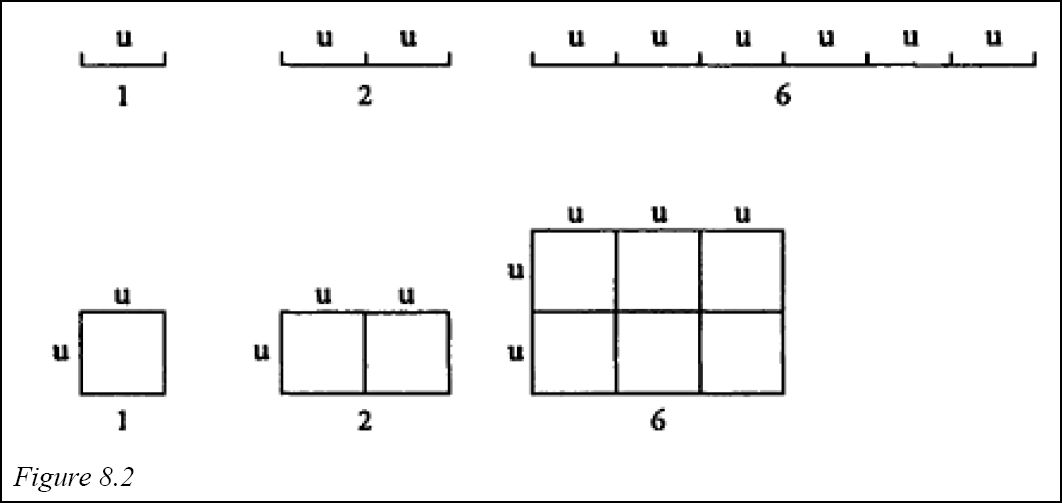). But this seems to be what lies behind the discussion in the Theaetetus. That is to say, it looks as though Theodoras was using a unit length u and proving what we would call the irrationality of n for certain √n by showing that the side sn of a square corresponding to n was incommensurable in length with u. Theaetetus made a generalization of what he was shown by Theodoras by assuming or proving that: (i) n is a perfect square if and only if sn is commensurable with u. It should be clear that there is a big difference between assuming and proving (i). And although the proof of implication from left to right is quite straightforward, the proof of right-left implication is far from it. In fact, it is just the proposition we would assert by saying that the square root of any non-square positive integer is irrational. There is no extant ancient proof of such an assertion. We may gain more insight by formulating the question raised by Theaetetus in the Theaetetus and his answer to it as: Question: If y is the geometric mean between m•u and n•u (i.e. if the square on y is equal to the rectangle on m•u and n•u), under what conditions is y commensurable with u? Answer: If y is the geometric mean between m•u and n•u, then y is commensurable with u (if and) only if y ≈ n•u, for some k. Phrased this way, Theaetetus’ problem is equivalent to looking at the geometric mean y between two commensurable straight lines x and z, and asking whether it is commensurable with the lines. One correct answer to this problem is the following: (ii) If y is the geometric mean between straight lines x and z, y is commensurable with x if and only if x has to z the ratio of a square number to a square number. (ii) is equivalent to Elements X.9, which a scholiast ascribes to Theaetetus: This theorem is the discovery of Theaetetus, and Plato recalls it in the Theaetetus, but there it is set out in a more particular way, here universally. For there squares which are measured by square numbers are said to also have their sides commensurable. But that assertion is particularized, since it doesn’t include in its scope all the commensurable areas of which the sides are commensurable. ([8.30] 5:450.16–21) If Theaetetus was interested in the commensurability of the geometric mean between commensurable straight lines with the straight lines, it does not seem unreasonable to suppose that he would also have considered the arithmetic and harmonic means between commensurable straight lines and seen right away that these are commensurable with the original lines.10 So we can imagine that Theaetetus showed that the arithmetic or harmonic mean between two commensurable straight lines is commensurable with the original lines, but that this holds for the geometric mean only in the case where the original lines are related as a square number to a square number; if they are not so related, he could only say that the geometric mean is commensurable in square with the original straight lines. He might now wonder whether, if we insert a mean x between lines y, z which are commensurable in square only, x is commensurable (at least in square) with y (and z). In fact this can be shown to hold for none of the means, and so we might imagine Theaetetus having proved: (iii) The insertion of any of the three means between incommensurable ‘rational’ lines produces an ‘irrational’ line. We might imagine him pushing on to further ‘irrational’ lines by inserting further means (cf. Elements X.II5), but I suspect that, if Theaetetus were looking to the notion of commensurability in square as a kind of limit on incommensurability, the recognition that any of the means between lines commensurable in square only goes beyond that limit might have given him pause. He would then have had a ‘theory’ summarized by (iii). This theory only gets us to the medial, not to the binomial and apotome. To explain the introduction of the binomial and medial we need only recall that the motivation for the whole theory of ‘irrational’ lines seems to be provided by the treatment of the regular solids in Book XIII.11 There remains the question of what exactly Theaetetus did and how it is related to Books X and XIII of the Elements. Pappus’ statement that Euclid formulated definitions for rationals and irrationals ‘and differentiae, determined also many orders of the irrationals, and brought to light whatever of definiteness is to be found in them’ suggests that quite a bit of book X is due to him. On the other hand, if one assumes, as it seems necessary to do, that Theaetetus’ interest in apotomes grew out of his study of the regular solids, then it seems plausible to assume that he established the characterizations of the edges of dodecahedron and icosahedron which we find in the Elements. But once we make that assumption it seems hard to deny that essentially all of X and XIII is due to Theaetetus,12 and that Euclid’s changes were more formal than substantive. However, we have no way of drawing an exact boundary between the work of the two men. (5) Theaetetus and the Theory of Proportion I have already alluded in section 1 to the major peculiarity involved in the treatment of proportionality in the Elements. Euclid gives one definition of proportionality for ‘magnitudes’ in Book V and another for numbers in Book VII. Then in X.5–8, with no indication that there is any problem, he introduces proportions involving both numbers and magnitudes. Understandably those who view the Elements as a loosely strung together compilation of independent treatises have focused considerable attention on this juncture in the text. A standard position is that (a) the ultimate sources of Book VII are chronologically earlier than the work of Eudoxus incorporated in Book V; (b) since Book X deals with incommensurable magnitudes, it obviously cannot be based on the theory of proportion of Book VII; and (c) since Book V has nothing to say about numerical ratios, Book X cannot be based on Eudoxus’s theory. What, then, could it be based on? This alleged gap in our knowledge was filled by Becker [8. 96], starting with a remark on definitions in Aristotle’s Topics (VIII.3.158b24– 35): Many theses are not easy to argue about or tackle because the definition has not been correctly rendered, e.g., whether one thing has one contrary or many…. It seems that it is also the case in mathematics that some things are difficult to prove because of a deficiency in a definition. An example is that a line which cuts a plane [i.e. parallelogram] parallel to a side, divides the line and the area similarly. For the assertion is immediately evident when the definition is stated. For the areas and the lines have the same antanairesis. And this is the definition of the same ratio. Commenting on this passage, Alexander of Aphrodisias says ([8.20], 545.15–17) that Aristotle calls anthuphairesis antanairesis and that early mathematicians called magnitudes proportional if they have the same anthuphairesis. In terms of Figure 8.3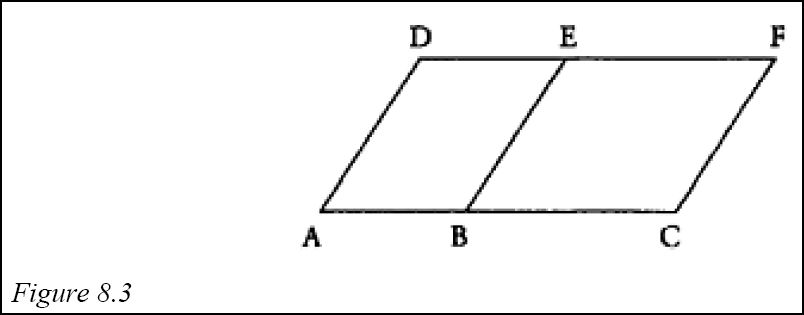Aristotle’s example of a proposition difficult to prove presumably says something like: Parallelogram ABED is to parallelogram BCFE as AB is to BC. In the Elements Euclid proves a similar result as VI.1. using the Book V definition of proportionality. Neither anthuphairesis nor antanairesis occurs with a mathematical sense in an ancient text outside the Topics passage and Alexander’s comment on it, but the verb anthuphairesthai is used by Euclid in the Elements, where Heath [8.32] translates it ‘be continually subtracted’ or ‘be continually subtracted in turn’. Two propositions in which this verb occurs are: VII.1. Two unequal numbers being set out and the less being continually subtracted in turn from the greater, if the number which is left never measures the one before it until a unit is left, the original numbers will be prime to one another. X.2. If, when the lesser of two unequal magnitudes is continually subtracted in turn from the greater, that which is left never measures the one before it, the magnitudes will be incommensurable. And the verb turns up in the proof of VII.2, which shows how to find the greatest common measure of two numbers, and in that of X.3, which shows how to find the greatest common measure of two commensurable magnitudes. The method used to find a common measure is what Alexander means by anthuphairesis. I illustrate its use to find the greatest common measure or divisor, 2, of 58 and 18: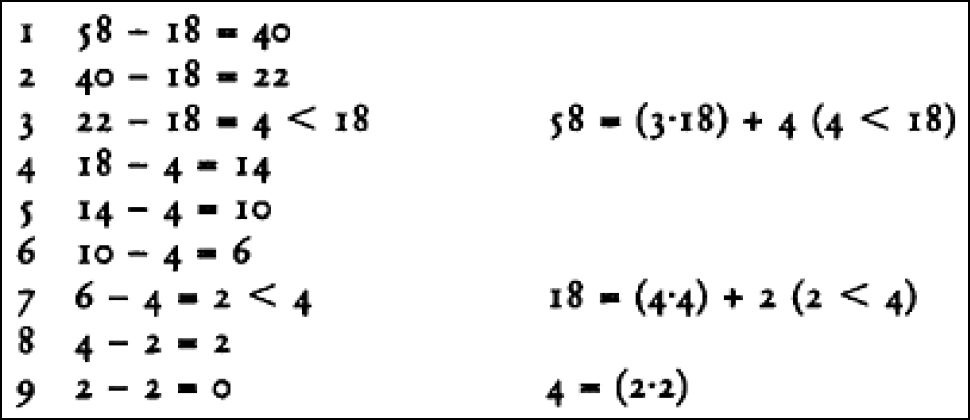I now give a general representation of the procedure for magnitudes x1 and x2 with x1>x2: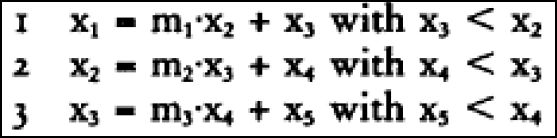This procedure either stops with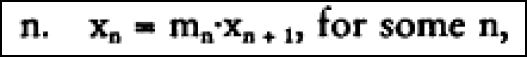or proceeds forever with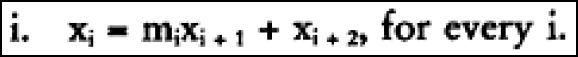Euclid takes for granted that, if we are dealing with numbers, the procedure will stop because eventually we will get 1 (the unit) as a remainder; we might say that in this case 1 is the greatest common measure, but Euclid normally distinguishes between 1 and a number. The argument that if the procedure stops at step n, xn+1 is the greatest common measure relies only on ideas about measuring, adding and subtracting, as does the argument that, if the procedure doesn’t stop, there is no common measure.13 The anthuphairesis of two quantities x1 and x2 is completely represented by the series of multipliers m1, m2…produced by the process of alternate subtraction. So presumably the definition of proportionality referred to by Aristotle says that two pairs of magnitudes are in proportion if the series produced by applying anthuphairesis to the first pair is the same as the series produced for the second. This definition obviously does apply to both numbers and to magnitudes. Since Aristotle refers to Eudoxus in several passages and the proportionality definition of Book V is ascribed to Eudoxus, there is reason to think that Aristotle is referring to a definition of the ‘early’ mathematicians, i.e. earlier than Eudoxus. Given other assumptions which we have already mentioned, it is a short step to ascribing the anthuphairetic definition of proportionality to Theaetetus and to supposing that the original of Book X was based on this definition. A crucial assumption for this view is the idea that there is a serious gap in the proofs of X.5–8. This claim is hard to evaluate because there are serious logical difficulties in Euclid’s treatment of proportionality in arithmetic itself.14 But if, as is generally done, those difficulties are ignored, and we assume that at least by the time of Book X Euclid includes numbers among magnitudes, then the only law which Euclid has not proved in Book V and which he needs to justify X.5–8 is trivial.15 The assumption that Euclid left the proof of this law to his readers or students does not seem to me implausible. However, even if this is true, Aristotle’s remark in the Topics makes it very likely that at some point before his and Eudoxus’ time an anthuphairetic theory of ratios was developed to apply to commensurable and incommensurable magnitudes.16 Whether we should ascribe this theory to Theaetetus seems to me moot. (6) Archytas, Harmonics and Arithmetic In section 4 I described the representation of the fundamental concords fourth, fifth and octave in terms of the ratios 4:3, 3:2, 2:1. In a fragment from On Mathematics (Porphyry [8.73], 56.5–57.27, DK 47 B 1), Archytas correlates high pitches with fast movements and low pitches with slow ones in a piece of physical acoustics.17 We find an analogue of this correlation in the prologue of Euclid’s Sectio Canonis, our earliest text in mathematical harmonics. The prologue concludes with an argument that it is ‘reasonable’ that: SC Assumption 1. The concordant intervals are ratios of the form n+1:n or n:1, i.e. they are either ‘epimorics’ or multiples. It seems possible that Archytas put forward an argument of the same kind, although only Euclid makes an explicit correlation between pitch and frequency.18 Euclid’s argument for SC Assumption 1 relies only on an analogy between the idea that concordant notes make a single sound and the fact that ratios of the two forms are expressed by a single name in Greek: double is diplasios, triple triplasios, etc., and 3:2 is hēmiolios, 4:3 epitritos, 5:4 epitetartos, etc. There is no question that the argument is a post hoc attempt to justify previously established correlations; and it fails rather badly as a foundation for the programme of the Sectio, which is: 1 to establish the numerical representation of the fundamental concords; 2 to use mathematics to disprove apparent musical facts, such as the existence of a half-tone; 3 to construct a diatonic ‘scale’. In the treatise Euclid tacitly takes for granted that addition of intervals is represented by what we would call multiplication of ratios, subtraction of intervals by what we would call division. To divide an interval represented by m:n in half is, then, to find i, j, k such that i:k :: m:n, and i:j :: j:k. In addition to SC Assumption 1 Euclid also relies on the following empirical ‘facts’: SC Assumption 2. fourth+fifth=octave. SC Assumption 3. fifth−fourth=tone. SC Assumption 4. The concords are in order fourth, fifth, octave, octave +fifth, and double octave. SC Assumption 5. Certain other intervals, in particular the double fourth and double fifth, are discordant and neither multiple nor epimoric. SC Assumption 5 brings out a kind of duplicity in the Sectio. In a (for him) ideal world Euclid would be able to say that all and only the concordant intervals are epimoric or multiple. To say that they all are would conflict with the fact that the octave+fourth (represented by 8:3) is concordant; Euclid passes over this interval in silence. To say that only they are would conflict with the fact that the tone (represented by 9:8 the ‘difference’ between 3:2 and 4:3) and the interval represented by 5:1 are discordant. Clearly Euclid picks and chooses his ‘facts’ as he needs them. Although the foundation of the Sectio is very nebulous and hardly what we would call scientific, and although SC Assumption 5 is used in a very arbitrary way, the core of the argumentation depends on quite sophisticated number theory. I shall not discuss the argumentation in detail,19 but do wish to mention three propositions of pure mathematics proved in the Sectio: SC Propositions 1, 2. If d1:d2 :: d2:d3, then d2 is a multiple of d1 if and only if d3 is. SC Proposition 3. If d1:d2 :: d2:d3 ::…:: dn−1:dn, then d1:dn is not epimoric. SC Proposition 2 enables one to establish the result that Plato ascribes to Theaetetus ((i) in section 4 above). In his proof of SC Proposition 2 Euclid justifies the crucial step by saying, ‘But we have learned that if there are as many numbers as we please in proportion and the first measures the last, it will also measure those in between.’ Euclid’s formulation here varies slightly from the formulation of the equivalent assertion as Elements VIII.7, a proposition which Euclid derives by reductio from its equivalent VIII.6: ‘If there are as many numbers as we please in continuous proportion and the first does not measure the second, none of the other numbers will measure any other.’ SC Proposition 3 is of special interest for fourth-century mathematics because Boethius ([8.28] III.11, DK 47 A 19) ascribes a similar proof to Archytas. To facilitate comparison of the two proofs I first give a simplified version of the Sectio proof: Suppose d1:dn is epimoric, and (i) let d+1 and d be the least numbers in the ratio of d1 and dn. (ii) No mean proportionals fall between d+1 and d, since no numbers fall between them at all. (iii) Therefore, there are no di such that d1:d2 :: d2:d3 ::… dn−1:dn. To justify this last step Euclid invokes the equivalent of Elements VIII.8 when he says, ‘However many means fall proportionally between the least numbers, so many will also fall proportionally between numbers having the same ratio.’ In Euclid’s actual argument step (i) is replaced by something like the following argument: (i′) Let d and d′ be the least numbers such that d′:d :: d1:dn. Then d′ and d have only the unit as common measure. Now consider d′−d; by the definition of ‘epimoric’ d′−d is a part of d and a part of d′; therefore it is the unit. In asserting that d′ and d have only the unit as common measure Euclid is apparently relying on the equivalent of VII.22 (‘The least numbers of those having the same ratio with one another are relatively prime’) and the definition of relatively prime numbers as those having only the unit as common measure (VII, def. 12). The proof ascribed to Archytas by Boethius is even messier. In place of (i′) it has: (i′′) Let d+d* and d be the least numbers such that (d+d*:d) :: d1:dn, so that, by the definition of ‘epimoric’, d* is a part of d. I assert that d* is a unit. For suppose it is greater than 1. Then, since d* is a part of d, d* divides d and also d+d*, but this is impossible. For numbers which are the least in the same proportion as other numbers are prime to one another and only differ by a unit.’ Therefore d* is a unit, and d+d* exceeds d by a unit. After inferring (ii′′) that no mean proportional falls between d+d* and d, Boethius concludes, presumably by reference to something like VIII.8: (iii′′) Consequently, a mean proportional between the two original numbers d1 and dn cannot exist, since they are in the same ratio as d+d* and d. In the quoted lines in (i′′) the equivalent of VII.22 is again cited, but, as Boethius points out, the words ‘only differ by a unit’ are not correctly applied to arbitrary ratios in least terms but only to epimoric ones.20 It seems reasonable to suppose that Archytas was responsible for something like the proof ascribed to him by Boethius, and that Euclid improved it in the Sectio, perhaps relying on the Elements as an arithmetical foundation. It even seems reasonable to suppose that Archytas composed some kind of Ur-Sectio, on which our Sectio was somehow based. However, it seems to me unlikely that our Sectio is simply an improved version of a work of Archytas. For Euclid’s diatonic scale is the standard one used by Plato in the Timaeus (35b–36a) for the division of the world soul into parts, whereas we know from Ptolemy ([8.77], 30.9–31.18, DK 47 A 16) that Archytas’s diatonic was quite different.21 The question whether Archytas’ tuning is mathematical manipulation without musical significance must be considered moot. Barker ([8.14], 46–52) has argued that musical practice may have played a much more significant role in Archytas’ theorizing than is usually allowed, but he leaves no doubt that a kind of mathematical a priorism, particularly the faith in the consonance of epimorics, played a central role in Archytas’ musical thought. Moreover, a passage in Porphyry ([8.73], 107.15–108.21, DK 47 A 17) suggests that Archytas used very arbitrary numerical manipulations to determine the relative concordance of octave, fifth, and fourth, subtracting 1 from each term of the corresponding ratios, adding the results for each interval and taking lower sums to mean greater concord; since, he declared the octave to be more concordant than the fifth, which, in turn is more concordant than the fourth. This mixture of mathematical reasoning and mathematical mystification makes it difficult for us to classify the musical work of Archytas (and even of Euclid) as either science or numerology.22 It is difficult to believe that Archytas did not know the truth of VIII.7 and 8, at least for the case of one mean proportional. But it is hard to see how he could even begin to think about such results without a well-developed idea of arithmetical reasoning and proof.23 PART TWO: THE SIXTH AND FIFTH CENTURIES (1) Thales and Early Greek Geometry In addition to his general remarks about Thales quoted in the introduction Proclus ([8.74]) records four of Thales’ mathematical achievements, twice citing Eudemus as authority. I quote the passages: (a) The famous Thales is said to have been the first to prove that the circle is bisected by the diameter. (157.10–11) (b) We are indebted to the ancient Thales for the discovery of this theorem [asserting the equality of the base angles of an isosceles triangle] and many others. For he, it is said, was the first to recognize and assert that the angles at the base of any isosceles triangle are equal, although he expressed himself more archaically and called the equal angles similar. (250.20–251.2) (c) According to Eudemus, this theorem [asserting the equality of the nonadjacent angles made by two intersecting straight lines]…was first discovered by Thales. (299.1–4) (d) In his history of geometry Eudemus attributes to Thales this theorem [asserting the congruence of triangles with two sides and one angle equal]. He says that the method by which Thales is said to have determined the distance of ships at sea requires the use of this theorem. (352.14–18, all four passages in DK 11 A 20) Other passages24 credit Thales with a method for determining the height of a pyramid by measuring its shadow and call him ‘the first to describe the right triangle of a circle’, whatever that may mean. However, the crucial passages are the four I have quoted. Dicks ([8.88], 302–3) seizes on the last to argue that Eudemus’ attributions to Thales are reconstructions which presuppose that Thales demonstrated in a basically Euclidean way geometrical theorems implicit in his more practical accomplishments. Even if one accepts the plausibility of this approach to ancient doxographical reports, two features of these particular ones may cause one to hesitate: the detailed point in (b) about Thales’ archaic vocabulary, and the fact that in (a) Thales is said to have proved something which is (illegitimately) made a matter of definition in Euclid’s Elements (I, def. 17). It is also striking that all four of the propositions ascribed to Thales can be ‘proved’ either by superimposing one figure on another (d) or by ‘folding’ a configuration at a point of symmetry. It seems possible that Thales’ proofs were what we might call convincing pictures involving no explicit deductive structure. But once one ascribes even this much of a conception of justification to Thales, one is faced with what would seem to be serious questions. How did it come about that Thales would formulate, say, the claim that a diameter bisects a circle? If he was just interested in the truth ‘for its own sake’, then we already have the idea of pure geometrical knowledge. But if, as seems more plausible, he was interested in the claim as a means to justifying some other less obvious one, then we seem to have the concept of mathematical deduction, from which the evolution of the concept of mathematical proof is not hard to envisage. We need not, of course, suppose that Thales was a rigorous reasoner by Euclidean standards; merely saying that he explicitly asserted and tried to justify mathematical propositions of a rather elementary kind is enough to give us a primitive form of mathematics. Of course, we would like to know something about the historical background of Thales’ interests. Proclus and other ancient sources give credit to the Egyptians, but modern scholars tend to be sceptical about these claims.25 Van der Waerden and others have invoked the Babylonians to fill the gap. I quote from his discussion of Thales ([8.13], 89), which shows that he also credits Thales with a high standard of mathematical argumentation. We have to abandon the traditional belief that the oldest Greek mathematicians discovered geometry entirely by themselves and that they owed hardly anything to older cultures, a belief which was tenable only as long as nothing was known about Babylonian mathematics. This in no way diminishes the stature of Thales; on the contrary, his genius receives only now the honor that is due it, the honor of having developed a logical structure for geometry, of having introduced proof into geometry. Indeed, what is characteristic and absolutely new in Greek mathematics is the advance by means of demonstration from theorem to theorem. Evidently, Greek geometry has had this character from the beginning, and it is Thales to whom it is due. (2) Harmonics in the Sixth and Fifth Centuries In his commentary on Ptolemy’s Harmonics ([8.73], 30.1–9), Porphyry says: And Heraclides writes these things about this subject in his Introduction to Music: As Xenocrates says, Pythagoras also discovered that musical intervals do not come to be apart from number; for they are a comparison of quantity with quantity. He therefore investigated under what conditions there result concordant or discordant intervals and everything harmonious or inharmonious. And turning to the generation of sound, he said that if from an equality a concordance is to be heard, it is necessary that there be some motion; but motion does not occur without number, and neither does number without quantity. The passage continues by developing an even more elaborate theory of the relationship between movements and sound than the ones I mentioned earlier in section 6. Scholars who are doubtful that Pythagoras was any kind of scientist are happy to deny that Heraclides is Heraclides of Pontus, the student of Plato, and to restrict the extent of the citation of Xenocrates to the first sentence.26 Even this sentence implies that Pythagoras discovered something about numbers and concords, and I think everyone would agree that, if he discovered any such thing, it was the association of the fundamental concords with the ratios 4:3, 3:2 and 2: 1. It is commonly thought that this association must have been known by people familiar with musical instruments quite independently of theoretical proclamations, but that ‘Pythagoras invested the applicability of these ratios to musical intervals with enormous theoretical significance’ [KRS p.235]. Burkert ([8.79], 374–5) has pointed out how difficult it is to identify an early instrument which would facilitate recognizing the correlation of pitch relations with numerical ratios. The traditional story of Pythagoras’ discovery of the ratios—for which our earliest source is Nicomachus ([8.57], 6)—depends upon false assumptions about the causal relation between pitches produced and the weights of hammers striking a forge or weights suspended from plucked strings. However we find a perfectly credible experiment, involving otherwise equal bronze discs with thicknesses in the required ratios, associated with the early Pythagorean Hippasus of Metapontum (Scholium on Phaedo 108d [8.64], 15, DK 18.12).27 Hippasus is thought to have flourished in the earlier fifth century. In an important sense he is our only clear example of a Pythagorean mathematical scientist before Archytas. But the stories about his relations to the Pythagoreans and the division of the school into akousmatikoi and mathēmatikoi surround him in a mysterious fog which is not fully penetrable.28 In section 4 I described the close relation of the doctrine of means with harmonics, and quoted the passage in which Archytas describes the three basic means. Proclus ([8.74], 67.5–6) indicates that Eudoxus added other means to the basic three. Nicomachus ([8.55], II.21) says that all the ancients, Pythagoras, Plato and Aristotle, agreed on the arithmetic, geometric and harmonic means. Iamblichus ([8.54], 100.22–4) says that Hippasus and Archytas introduced the name ‘harmonic’ in place of ‘subcontrary’, and in two passages ([8.54], 113.16– 17, 116.1–4) he associates the introduction of additional means with Hippasus and Archytas. Whether or not the additional means can be ascribed to Hippasus, it seems plausible to suppose that he did work with ratios and at least the first three means in the earlier fifth century. His doing so certainly implies some level of mathematical abstraction and manipulation, but presumably the level might be fairly low. We do not gain much clarification in this matter when we turn to the other main allegedly fifth-century treatment of mathematical harmonics, which is ascribed to Philolaus. In the second part of DK 44 B 6 (put together from two versions, Stobaeus ([8.86] I.21.7d) and Nicomachus ([8.57], 9)), Philolaus constructs an octave with seven tones, the first four of which quite clearly form a tetrachord in the standard diatonic system (see note 21). In his own vocabulary he mentions the ratios for the three fundamental concordant intervals, and asserts the following: fifth−fourth=9:8; octave=five 9:8 intervals+two ‘dieses’; fifth=three 9:8 intervals+one ‘diesis’; fourth=two 9:8 intervals+one ‘diesis’. Boethius ([8.28] III.8, DK 44 B 6) tells us that for Philolaus the ‘diesis’ or smaller semitone is the interval by which 4:3 is greater than two tones, so that there is no reason to doubt that Philolaus has the mathematics of the standard diatonic scale. However Boethius goes on to say that the ‘comma’ is the interval by which 9:8 is greater than two ‘dieses’, and that the ‘schisma’ is half of a ‘comma’, and the ‘diaschisma’ half of a ‘diesis’. The ‘diesis’ should be 256:243 and the ‘comma’ 531441:524288. Neither of these intervals can be divided in half in the sense of the Sectio Canonis. Since Philolaus seems clearly to recognize that the tone cannot be divided in half, it is rather surprising that he apparently takes for granted—what is false in terms of the Sectio— that there are half ‘dieses’ and half ‘commas’. But the situation becomes even more problematic when one takes into account III.5 of De Institutions Musica (DK 44 A 26). For there Philolaus garbles together the combining and disjoining of ratios with the adding and subtracting of numbers. He also moves without comment from taking an interval as a ratio between two numbers m and n and as their difference m−n. He begins by taking 27 as the cube of the first odd number, and then expresses the tone (9:8) as 27:24. He says that this is divisible into a larger and smaller part, the ‘apotome’ and the ‘diesis’, the difference between them being a ‘comma’.29 Taking the standard value for the ‘diesis’, 256:243, he treats it as if it were 13 (=256−243), pointing out that 13 is the sum of 1 (‘the point’), 3 (‘the first odd line’) and 9 (‘the first square’). To find the ‘apotome‘ he uses the value 243:216 (9:8) for the tone and says that 27 (=243−216) is the tone. The value of the ‘apotome’ is then 14 (=27 −13) and the value of the ‘comma’ is 1 (=14−13). This discussion is, of course, pure nonsense. For Burkert the nonsense is genuine late fifth-century Pythagoreanism, which ‘shows a truly remarkable mixture of calculation and numerical symbolism in which ‘sense’ is more important than accuracy’ ([8.79], 400). For Huffman ([8.61], 364–80), whose Philolaus and fifth-century Pythagoreanism are much more scientific than Burkert’s, just the description of the seven-note scale with the diatonic tetrachord is genuine Philolaus. I remark only that everywhere in what we might call the Pythagorean tradition of Greek music, including Archytas, Plato, Euclid and Ptolemy, the sense of the cosmic power of pure numbers and the willingness to indulge in meaningless numerical manipulation is always present. What distinguishes Philolaus, from Euclid and Ptolemy certainly, and for the most part from Archytas as well, is the apparent confusion between numerical relations or ratios and absolute numbers. Even if we waive the question of authenticity, I do not think there is sufficient evidence to decide whether Philolaus represents the sort of thing one would expect of any fifth-century Pythagorean. But there is little doubt that it can be expected of some. (3) Arithmetic in the Sixth and Fifth Centuries It is customary to associate the representation of the fundamental concords as ratios with an important concept of Pythagorean lore, the tetraktus, the first four numbers represented by the triangle of Figure 8.4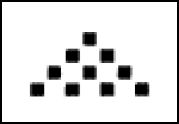and summing to 10, the perfect number encapsulating all of nature’s truth.30 In II.8 of his Introduction to Arithmetic Nicomachus introduces the notion of a triangular number, that is a number which can be represented in triangular form, as in Figure 8.5.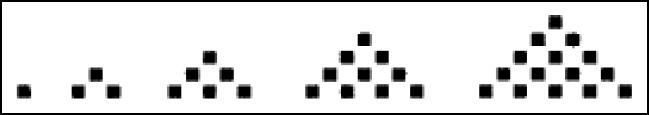It is clear that the triangular numbers form an infinite sequence and that the nth triangular number is the sum of the first n numbers.31 I shall call these arrays of dots figurate numbers. In succeeding chapters Nicomachus describes square numbers, pentagonal numbers, and so on up to octagonals. In his commentary on Nicomachus’s presentation of triangular numbers Iamblichus says ([8.54], 58.19– 25; cf. Aristotle, Categories 14.15a29–33) that the number added to the mth nagonal number to get the m+1th is called the gnomon, the thing which preserves the shape of a thing when added to it. He explains that the term was taken from geometry, where it was applied to the excess by which one square exceeds another. Figure 8.6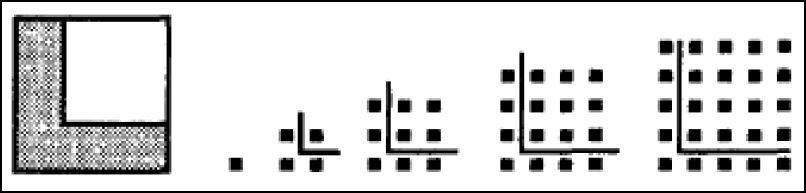shows what he means and how the gnomon functions in the generation of square numbers.32 It makes quite clear that the nth square number is the sum of the first n odd numbers, one example of the way in which relatively simple manipulation of figurate numbers can establish mathematically interesting results independently of anything resembling a stylized Euclidean deduction. But the difference between such deduction with its definitions, technical vocabulary, diagrams and formalistic descriptions, on the one hand, and informal manipulation designed to bring out general truths about numbers or rules for producing them is not great. Nor are the moves from the sacred tetraktus to triangular numbers to generalizations about them and other polygonal numbers. Our evidence for figurate numbers is late, Theon of Smyrna, Nicomachus, and Iamblichus being the principal sources. There is no trace of figurate numbers in Euclid. Nevertheless, most scholars take the material in Theon, Nicomachus and Iamblichus to be early. In his History Heath discusses this material under the rubric ‘Pythagorean arithmetic’ before he discusses Thales. I do not wish to suggest that his doing so is illegitimate, but only to insist that once one admits an interest, even a numeromystico-theological interest, in accumulating general numerical laws and rules on the basis of the manipulation of configurations of dots, one has the fundamentals of a scientific arithmetic, although not, of course, an arithmetic in which one advances ‘by means of demonstration from theorem to theorem’, to use van der Waerden’s description of the geometry of Thales.33 As an example of the power of the manipulation of figurate numbers, I want to consider the so-called Pythagorean theorem (Elements I.47). It is now a commonplace of mathematical history that the theorem is not the discovery of Pythagoras,34 but was known by the Babylonians centuries before he was born. One need not, however, suppose that Greek knowledge of the theorem came ultimately from Babylonia. The primary Greek account of Pythagoras’s discovery of the theorem35 caused even his later admirers difficulty because in it the allegedly vegetarian Pythagoras was said to have celebrated his discovery by sacrificing an ox. For example, Proclus ([8.74], 426.5–9) says: If we listen to those who like to give an account of old things, one will find them attributing this theorem to Pythagoras and saying that he sacrificed an ox on its discovery. Proclus then immediately turns to praise for Euclid for generalizing the theorem from squares to similar figures (Elements VI.31). Proclus also attributes to Pythagoras a procedure for generating numbers satisfying the theorem;36 the procedure starts with an odd number m and takes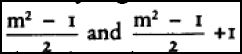Heath ([8.7]: 80) shows how this rule could be related to the generation of the square numbers through the addition of gnomons. It is clear from Figure 8.6that the square number (n+1)2 is generated from a square number n2 by the addition of the gnomon 2n+1; but if 2n+1=m2, n=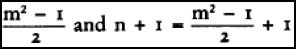. Becker  pointed out an odd feature of the last sixteen propositions (IX.21– 36) of the arithmetic books of the Elements. In IX.20 Euclid proves one of the old chestnuts of arithmetic, the infinity of the prime numbers. In IX.21 Euclid proves on the basis of definitions only that the sum of any number of even numbers is even; and there follows a string of other relatively elementary propositions. However, the string culminates in another old chestnut of arithmetic (IX.36), that if p=20 + 21+22…+2n and is prime, p•2n is perfect, i.e. equal to the sum of its factors other than itself.37 Euclid’s proof of this result uses propositions proved before IX.21 and none from IX.21–34. IX.21–34 are, with the exception of 32, a self-contained deductive sequence dependent only on definitions. Becker argued that the propositions in the sequence could all be proved on the basis of figurate numbers if one understood the product of two numbers to be a rectangle with the numbers as ‘sides’ (see Figure 8.7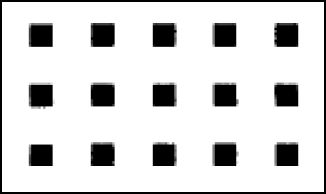, which represents the product of 3.5), and understood even and odd in the way they are defined in the Elements, where an even number is said to be one which is divisible into two equal parts and an odd to be one which is not even or which differs from an even number by 1 (VII, defs 6 and 7). Becker also showed that 36 could be incorporated into the sequence and proved on the same basis, eliminating the need for IX.35, which Euclid proves as a lemma for 36. Becker’s claim that he had reconstructed a piece of early Pythagorean deductive arithmetic has won considerable, although not universal, acceptance among historians of Greek mathematics. Subsequently Becker ([8.1], 41, [8.2], 51–2) offered a proof of the same kind for what he called the irrationality of 2, but which we can think of as the claim that there is no square number which added to itself produces a square number. Clearly if a Pythagorean was interested in finding numbers satisfying the Pythagorean theorem in general, he might have been interested in finding numbers satisfying it in the particular case of isosceles right triangles. Like the justification of Pythagoras’ rule for finding numbers satisfying the Pythagorean theorem, the proof begins by imagining what things would be like if such numbers were found. It is easy to see (Figure 8.8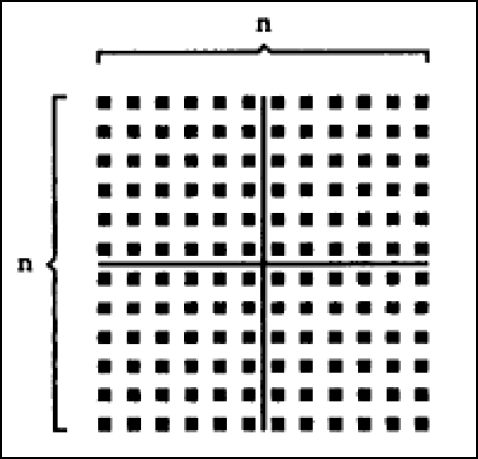) that the square of an even number is even and, in fact, divisible into 4 equal square numbers. But if n2=2m2, then n2 is even by definition and the left and right halves of the figure are equal to m2, which itself is divisible into two square numbers, each equal to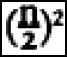. Clearly then m2 is a smaller number than n2 and is twice a square number, which is twice a smaller square number m1 2, which obviously will be twice a smaller square number m2 2, and so on ad infinitum. But such an infinite sequence of smaller numbers is impossible. Becker’s reconstruction is a reconstruction. There is no evidence to support the claim that there ever were arguments of the kind that he presents, let alone that they were given by early Pythagoreans. Burkert ([8.79], 434–7) raises a number of objections to the reconstruction, of which I mention two.38 The first is that the reconstructed theory has a deductive structure, but the Pythagoreans ‘did not deduce one proposition from the other’. The second is that the Pythagoreans, being ‘simpler souls’ would be satisfied with seeing inductively that there are no configurations satisfying the equation n2=2m2 and could not proceed by imagining a configuration satisfying it. Both of these assertions seem to me to beg important questions. It may well be a mistake to assign Euclidean formality to the early fifth century, but there doesn’t seem to me anything conceptually or psychologically difficult in imagining that Pythagoreans who showed that, say, the sum of two even numbers is even by using figurate numbers might show that an even plus odd number is odd by pointing out that an even plus an odd number is an even number plus an even number plus 1. Nor does it seem to me difficult to imagine a Pythagorean simple soul who has seen ‘inductively’ that no n2 is 2m2 using a picture like Figure 8.8to demonstrate the fact, while ignoring the point that the figure ‘really’ shows only that 122=72+72 and does not represent 72 as a square number. (4) Geometry in the Sixth and Fifth Centuries Application of areas Proclus’s general description of Pythagoras’ contributions to geometry has a blatantly Neoplatonic sound. Burkert ([8.79], 409–12) has invoked a partial similarity to a sentence of Iamblichus ([8.51], 70.1–7) to argue that Proclus’ entire description of Pythagoras with the ascription to him of the study of irrationals and the construction of the regular solids—for Proclus the goal of the Elements as a whole—is unreliable. In section 3 of pan one I pointed out that Theaetetus probably was the first person to treat the five regular solids in a roughly systematic way. Before discussing irrationality I want to mention a passage in which Proclus cites Eudemus for an ascription, not to Pythagoras, but to the ‘ancient’ Pythagoreans.39 In Elements I.44 Euclid shows how, given a straight line AB, a triangle b, and an angle EFG, to construct a parallelogram ABCD equal to b and with angle DAB equal to angle EFG (See Figure 8.9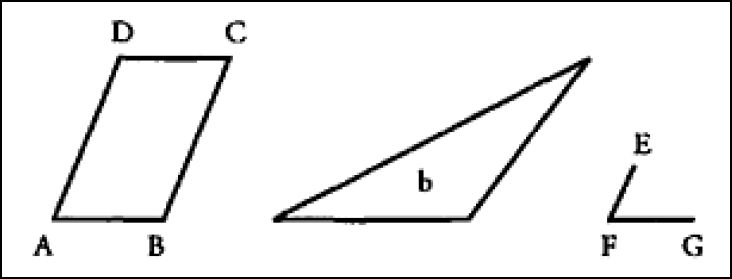). At the beginning of his discussion of this proposition Proclus ([8.74], 419. 15– 18) writes: Those around Eudemus say that the following things are ancient and discoveries of the muse of the Pythagoreans: the application [parabolē] of areas and their excess [hyperbolē] and their deficiency [elleipsis]. In I.44 the given area b is ‘applied’ to the straight line AB. In VI.28 (VI.29) Euclid shows how, given a rectilineal figure b (b′) and a parallelogram EFGH, to apply to a straight line AB a parallelogram AB′C′D (AB′′C′′D) which is equal to b (b′) and ‘deficient’ (‘excessive’) by a parallelogram BB’C′C (BB′′C′′C) similar to EFGH (See Figure 8.10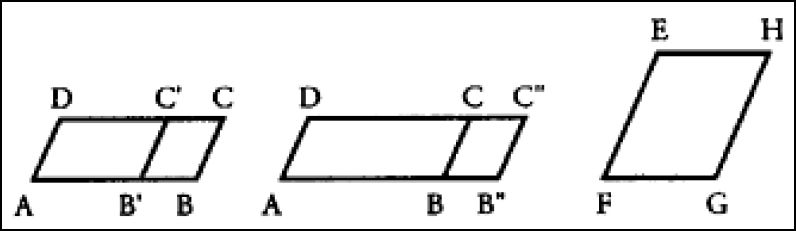).40 In his headnote to Book II of the Elements Heath ([8.32] 1:372) writes: We have already seen how the Pythagoreans and later Greek mathematicians exhibited different kinds of numbers as forming different geometrical figures. Thus, says Theon of Smyrna (p. 36, 6–11), ‘plane numbers, triangular, square and solid numbers, and the rest are not so called independently…but in virtue of their similarity to the areas which they measure; for 4, since it measures a square area, is called square by adaptation from it, and 6 is called oblong for the same reason’. A ‘plane number’ is similarly described as a number obtained by multiplying the two numbers together, which two numbers are sometimes spoken of as ‘sides’, sometimes as the ‘length’ and ‘breadth’ respectively of the number which is their product. The product of two numbers was thus represented geometrically by the rectangle contained by the straight lines representing the two numbers respectively. It needed only the discovery of incommensurable or irrational straight lines in order to represent geometrically by a rectangle the product of any two quantities whatever, rational or irrational; and it was possible to advance from a geometrical arithmetic to a geometrical algebra, which indeed by Euclid’s time (and probably long before) had reached such a stage of development that it could solve the same problems as our algebra so far as they do not involve the manipulation of expressions of a degree higher than the second. (Cf. van der Waerden [8.13], 124) Heath here presents what I will call the algebraic interpretation of Greek mathematics, an interpretation he takes over from Tannery and Zeuthen.41 We can get a good enough sense of what he has in mind by considering the propositions I used to illustrate application of areas. These propositions are given their ‘algebraic’ sense when, as Heath’s remarks suggest, the angle EFG is taken to be right, the parallelogram EFGH to be a square, and the other parallelograms to be rectangles. For then the area b represents a known quantity b and the straight line AB represents a known quantity a. In I.44 AD represents the value x which solves the equation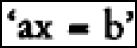.42 And in VI.28 (VI.29) the straight line BB ′ (BB′′) represents the solution of the equation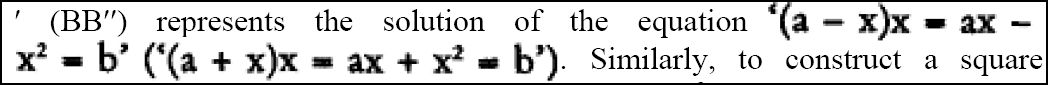. Similarly, to construct a square equal to a given rectilineal figure (II.14) is to solve ‘x2=b’. It is important to see that the algebraic interpretation of an important part of Greek mathematics involves treating its geometric form as mere form. The qualitative geometric character of Greek mathematics becomes a mask for a quantitative and calculational notion of ‘what’s really going on’. It is in large part because of this picture that Heath moves Pythagorean arithmetic to the front of his History, preceding it by a chapter on ‘Greek numerical notation and arithmetical operations’, a chapter which likewise relies entirely on late Greek sources. Tannery, Zeuthen and Heath all did their work before the decipherment of Babylonian materials. These materials provided the basis for an interpretation of Babylonian mathematics as an algebra which solves numerical problems. With this discovery people were in a position to argue that the geometrical clothing which the Greeks allegedly put on their ‘algebra’ was sewn out of Babylonian cloth to clothe a Babylonian body.43 And the answer to why the Greeks bothered with all the fancy tailoring was found in their deductive rigour and the discovery of incommensurability. I quote van der Waerden ([8.13], 125–6): In the domain of numbers the equation x2=2 cannot be solved, not even in that of ratios of numbers. But it is solvable in the domain of segments; indeed the diagonal of the unit square is a solution. Consequently, in order to obtain exact solutions of quadratic equations, we have to pass from the domain of numbers to that of geometric magnitudes. Geometrical algebra is valid also for irrational segments and is nevertheless an exact science. It is therefore logical necessity…which compelled the Pythagoreans to transmute their algebra into a geometric form.44 Thus we have a complicated story of (a) Greek borrowing of Babylonian computational mathematics, (b) discovering incommensurability, and (c) developing a rigorous geometric disguise for carrying on with Babylonian computation. If we assume that when Eudemus says that the discovery of application of areas was ancient and Pythagorean, he intends to refer to what we call early Pythagoreans, the whole history has to be moved back at least to the early fifth century. The way Burkert ([8.79], 465) avoids this difficulty is to leave the word ‘ancient’ out of account and argue that the discovery of incommensurability is ‘not far from Theodoras of Cyrene’,45 and hence to make (c) apply to ‘late’ Pythagoreans. My preference is to give up the whole idea that Greek mathematics is essentially computational and hence the idea that it rests on Babylonian achievements. With this point of view the question of the discovery of incommensurability becomes independent of positions on the nature and origins of Greek mathematics and can be approached on its own. I wish I could say that approaching the question this way made one answer or another probable, but, if we abandon Proclus’ statement about Pythagoras, the evidence for dating is unsatisfactory. The terminus ante quem is provided by references to irrationality in Plato; and if we believe that the mathematics lesson of the Theaetetus gives an indication of the state of mathematical knowledge in the 410s, we will be struck by the fact that Theodoras starts his case-by-case treatment with 3, and not 2; we might, then, take 410 as the terminus. The discovery of incommensurability: Hippasus of Metapontum The version of the Becker proof of the irrationality of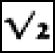which I presented in section 2 of this part is just a reformulation of the argument to which Aristotle refers (Prior Analytics I.23.41a26–7) when he illustrates reductio ad absurdum by referring to the proof that ‘the diagonal of the square is incommensurable because odd numbers become equal to evens if it is supposed commensurable’. A version of this proof occurs in our manuscripts as the last proposition of Elements X, but is printed in an appendix by Heiberg ([8.30] 3:408.1ff.). That version differs from the proof I gave in avoiding direct reference to the impossibility of an infinitely descending sequence of numbers by assuming that n and m are the least numbers such that n2=2m2 and inferring that, since n is even, m must be odd; but then the argument shows that m must be even. It is frequently assumed that some such proof was the first proof of incommensurability, partly because of the passage in Aristotle and partly because side and diagonal of a square are the standard Greek example of incommensurability. In its Euclidean form the proof presupposes a fairly sophisticated understanding of how to deal with ratios in least terms—an important subject of Elements VII, which is dependent on anthuphairesis. There are various ways of minimizing this presupposition, but in general those who believe that the Euclidean proof is a version of the original proof have used its relative sophistication to argue either that the proof must be late or that Greek mathematics must have been sophisticated relatively early. Von Fritz [8.46] ascribed the discovery of incommensurability to the Pythagorean Hippasus of Metapontum on the basis of two texts of Iamblichus printed under DK 18.4. The first says: About Hippasus they say that he died at sea for impiety because he published and described the sphere composed of twelve pentagons [i.e the dodecahedron] and allowed himself to be credited with the discovery, but all these things were the discoveries of ‘that man’ (for this is the way they refer to Pythagoras and not by his name). Mathematics advanced because of these things, and two people were most of all considered the first mathematicians of the time, Theodoras of Cyrene and Hippocrates of Chios. (Iamblichus [8.53] 78.27–36) In the other text Iamblichus ([8.51] 132.11–23) does not mention Hippasus, but says that the divine destroyed at sea the person who revealed the construction of the dodecahedron. Iamblichus adds that ‘some people say it was the person who spoke out about irrationality and incommensurability who suffered this’. Von Fritz claimed that Hippasus discovered irrationality in connection with the regular pentagon (the face of the dodecahedron) and the star or pentagram, a Pythagorean symbol formed by connecting alternating vertices of the pentagon. I sketch, with reference to Figure 8.11, the reasoning von Fritz ascribed to Hippasus.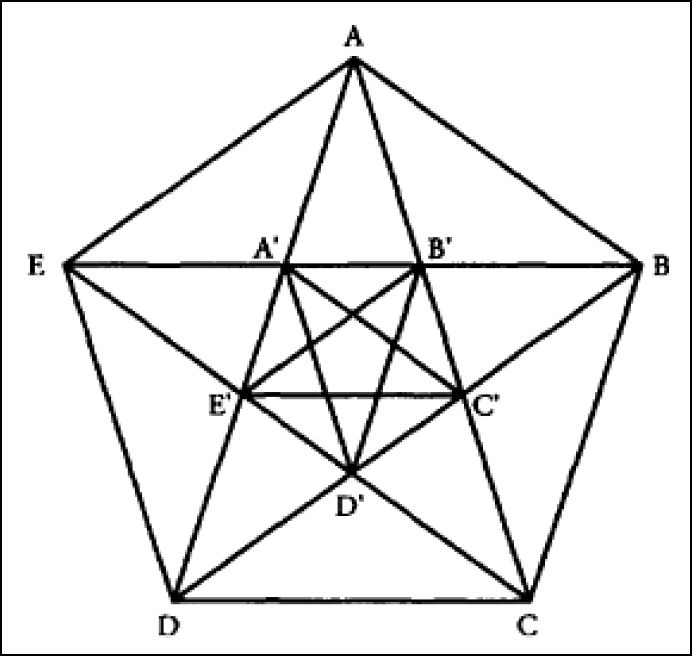Suppose one tries to find the greatest common measure of the side AE and the diagonal AD. It is clear that AE≈AE′, so that AD −AE≈E′D. But E′D<AE and E′ D≈AA′. Hence when E′D measures AE′ it leaves A′E′ as a remainder. But now E ′D≈D′D≈ A′D′, so performing anthuphairesis on A′E′ and E′D is the same as performing it on A′E′ and A′D′, i.e. on the side and diagonal of the regular pentagon A′B′C′D′E′. Clearly, the initial situation repeats itself and will repeat itself infinitely often as anthuphairesis is continued. Hence diagonal and side have no common measure. A Euclidean version of this argument would, of course, require justifications of the equalities and inequalities educed. One might assume that Hippasus could and would give such justifications. But, like Beeker’s arguments with the number configurations, Hippasus’s alleged argument could be a verbalization of something which is ‘seen’ to be true from the figure. However, the Hippasus argument, like the proof using the expression of ratios in least terms, presupposes the use of anthuphairesis to find a common measure,46 and hence— using the correlation between commensurability and having the ratio of a number to a number—the desire to express quantitative relations numerically. This desire is a primary mathematical component of numerous scholarly representations according to which the early Pythagoreans were committed to a view that ‘everything is number’, sought to find numerical expressions for all kinds of relationships, and were thrown into a crisis by the discovery of incommensurability.47 The metaphysical component of these representations of early Pythagoreanism has, I think, been largely discredited, as have the attempts to connect Zeno’s paradoxes with the issue of incommensurability.48 And so perhaps has the idea that the discovery of incommensurability caused some kind of crisis (as opposed to a difficulty requiring a solution) in mathematics itself. But, as we have seen, the view that Greek mathematics was importantly numerical and calculational and that the discovery of incommensurabilty ‘forced’ it to become geometricized remains an anchor of many contemporary presentations of Greek mathematics. However, the information which we have is more than merely compatible with the view that the Greeks pursued arithmetic and geometric investigations, including the application of areas, at a reasonably early time and that they continued to do so after the discovery of incommensurability. The question of how rigorous early Greek mathematics was seems to me quite unanswerable. If we accept the reports on Thales’ geometric accomplishments at more or less face value, we are presumably committed to saying that some idea of proof was functioning in Greek mathematics at a very early stage. If we do not, we are in no position to make any definite statement about its emergence, except that it did emerge. There are analogues of mathematical argument in Parmenides’ deduction and in Zeno’s dialectical argumentation. But the direction of influence seems to me quite indeterminable. I make no more than my guess in saying that I don’t believe that mathematical argument was influenced by either one of them49 and that, whereas Parmenides’ argumentation looks to be autonomous and satisfactorily explained without invoking mathematical precedent, Zeno’s considerations of infinite divisions seem likely to reflect mathematical preoccupations. The later fifth century: the quadrature of the circle In his history Proclus moves directly from Pythagoras to Anaxagoras, Oinopides of Chios, Hippocrates of Chios, and Theodoras of Cyrene. After his brief remark on the advancement of mathematics because of Hippasus’ mathematical revelations, Iamblichus mentions only the last two of these people ([8.53], 78.27– 36, quoted in the previous section). We know essentially nothing about the mathematical accomplishments of Anaxagoras,50 although Plutarch (On Exile 607F, DK 59 A 38) tells us that he managed to square the circle while in prison. From passages in Aristotle and comments on them we learn of other apparent attempts to square the circle by Antiphon (late fifth century), Bryson (fourth century), and Hippocrates of Chios.51 Antiphon apparently argued that one of the successively larger inscribed polygons of a sequence like the one indicated in Figure 8.1would coincide with the circumscribing circle, and Bryson that, since there is a square larger than a given circle and a square smaller than it, there is one equal to it. From the point of view underlying Eudoxus’ method of exhaustion Antiphon’s argument would seem to ignore the difference between arbitrarily close approximation and coincidence. Bryson’s argument is not a fallacy, but establishes (on the basis of some intuition about continuity) only the existence of a square equal to a given circle without showing how to construct it. Hippocrates and Oinopides Hippocrates’ reasoning has been the subject of considerable discussion because Simplicius’ presentation of his argument, based on the account of Eudemus, is our fullest representation of a piece of fifth-century mathematics. In the Physics (I.2.185a14–17) Aristotle refers to a quadrature by means of segments as if it made an incorrect inference from true geometrical principles. In the Sophistical Refutations (11.171b13–18) he refers to a false proof of Hippocrates in a context in which he also mentions Bryson’s quadrature and a quadrature by means of lunes, that is, plane figures contained by two circle arcs such as the darkened areas in Figure 8.12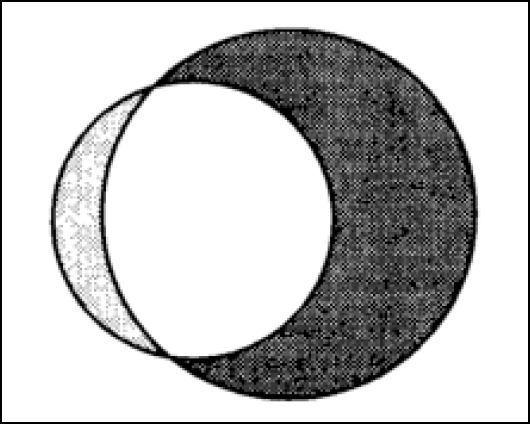. Subsequently (171b38–172a7) Aristotle characterizes the quadrature by means of lunes in much the way that he characterized the quadrature by means of segments in the Physics. The ancient commentators on the Physics passage, starting with Alexander, all take the quadrature by means of segments to be the quadrature by means of lunes and to be the work of Hippocrates. In his comment on the Physics passage ([8.84], 60.27–30) Simplicius invokes Eudemus: I will set out precisely what Eudemus says, but for the sake of clarity I will add a few things taken from Euclid’s Elements, because Eudemus comments in the old-fashioned way and sets out explanations in abbreviated form. Obviously our perception of Hippocrates’ reasoning is mediated by both Simplicius and Eudemus. We might suppose that, once the quotations from Euclid have been subtracted, the remainder of the material which follows is by Eudemus, but that still leaves us with the question of distinguishing Eudemian from Hippocratic material, a question which does not seem to be capable of being settled.52 Simplicius’ extract from Eudemus begins as follows: In the second book of his history of geometry Eudemus says the following. The quadratures of lunes, which are not considered as superficial constructions (diagrammata) because of their connection with the circle, were first described by Hippocrates in a way which was considered to be in order. Let us therefore touch on this subject in more detail and go through it. He made himself a starting-point and set out as the first of the things useful for the quadratures, the proposition that: (i) similar segments of circles have to one another the same ratio as their bases have in square. He showed this on the basis of having shown that: (ii) diameters [of circles] have the same ratios in square as the circles do, a proposition which Euclid puts second in Book XII of the Elements, where the proposition says ‘Circles are to one another as the squares on their diameters’. For as the circles are to one another, so are their similar segments, since: (iii) similar segments are those which are the same part of a circle, for example, a semicircle is similar to a semicircle, a third of a circle to a third of a circle. Therefore, (iv) similar segments admit equal angles, at least the angles of all semicircles are right, and the angles of segments greater than semicircles are less than right angles and as much less as the segments are greater than semicircles, and the angles of segments less than semicircles are greater than right angles and as much greater as the segments are less than semicircles. ([8.84], 60.30–61.18, my numbers inserted) Proposition (ii) is, as Simplicius says, equivalent to Elements XII.2, which is proved by the method of exhaustion (see section 1 of part one). Simplicius says that Hippocrates ‘showed’ (deiknumi) (ii), but scholars are reluctant to admit that he could have proved it in a rigorous fashion. Euclid takes (iv) as the definition of similar segments (III, def. 11), but he himself makes very little use of them. Hippocrates apparently defined two segments to be similar if they are the same ‘part’ of the circles of which they are segments. In Euclid a part of something is one nth of it, which would mean that Hippocrates picked out very few of the similar segments, indeed, none greater than a semicircle, and certainly none of the incommensurable ones. The way in which Hippocrates gets from (iii) to (iv) suggests that he wasn’t using much of a notion of proportionality at all, and just arguing in some sort of loose way. Obviously this looseness bears on the question whether or not Hippocrates knew about incommensurability. Again we are faced with standard kinds of choice: blame Simplicius or Eudemus for misunderstanding; assume that Hippocrates’ argumentation was not entirely rigorous; assume that incommensurability was discovered after Hippocrates squared his lunes or at least not long before. Finally, the move from (ii) to (i) seems to require some proposition about the relationship between the base of a segment of a circle and the diameter of the circle, perhaps that the segment is to the semicircle as the square on its base is to the square on the diameter. But we are not told how Hippocrates might have proved this. Hippocrates squared in succession three particular lunes, one with a semicircle as outer circumference, one with an outer circumference greater than a semicircle, and one with an outer circumference less than a semicircle, and then a circle plus a particular lune. Simplicius’ report does not make it seem as though Hippocrates claimed to have shown how to square the circle because he had shown how to square a circle plus a lune and how to square lunes with an outer circumference of ‘any size’. Perhaps he did, but it seems equally likely that the investigations described by Simplicius were an attempt at quadrature which somehow was interpreted to involve a claim to success. It is not possible for me to describe here Hippocrates’ four quadratures.53 I shall, however, mention one construction described by Simplicius. In the left configuration in Figure 8.13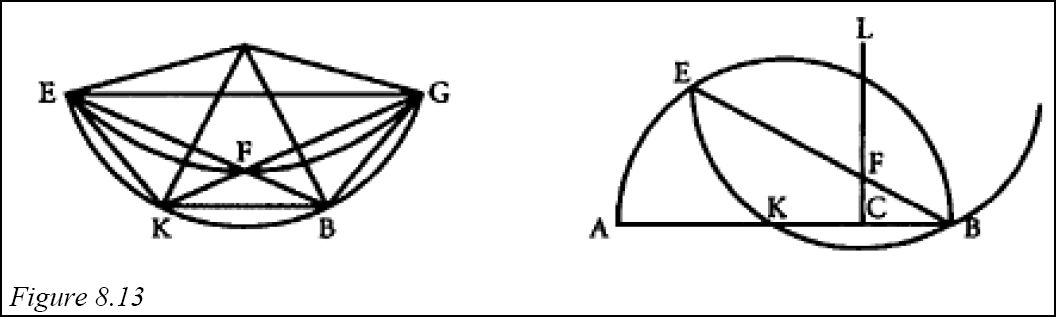, EG is parallel to KB, EK≈KB≈BG, EF≈FG, and the circle segments on EF, FG, EK, KB and BG are all similar. It should be clear that the lune EKBGF will be squarable if: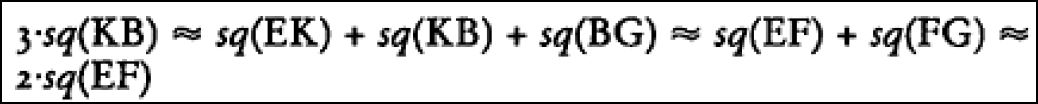since then the circle segments on EK, KB and BG are together equal to the circle segments on EF and FG and the lune EKBGF is equal to the rectilineal figure EKBGF. The right configuration in Figure 8.13 shows how Hippocrates manages to produce this result. He starts from a semicircle AKCBE with centre K, and lets CL be the perpendicular bisector of KB. He then finds F on CL such that the continuation of BF intersects the semicircle at E with 2•sq(EF)≈3•sq(KB). It is then a simple matter to carry out the rest of the construction. What our text doesn’t tell us is how Hippocrates proposed to find F. This could be done by a socalled verging argument (neusis). There is no problem in constructing a straight line E′F′ satisfying 2.sq(E′F′)≈3•sq(KB). One might think of the verging argument as a matter of marking E′F′ on a line (or ruler) and then moving the line around until a position is found in which E′ lies on the circumference, F′ on CL, and the line passes through point B. However, the problem can also be solved by a fairly complicated application of areas. It seems reasonable to suppose that the original Hippocratean material from which Simplicius’ report ultimately derives represented a high standard of geometric argumentation. Since Proclus tells us that Hippocrates was the first person said to have written elements, it also seems reasonable to suppose that at least parts of Hippocrates’ geometric work were built up in something like the Euclidean way. Hippocrates’ interest in mathematical methodology is borne out by another of his accomplishments, his reduction of the problem of constructing a cube twice the size of a given one to the finding of two mean proportionals between two given straight lines x and y, that is finding z and w such that x:z :: z:w :: w:y (Eutocius [8.44], 88.17–23, DK 42.4). According to Proclus ([8.74], 212.24–213.11), Hippocrates was the first person to ‘reduce’ outstanding geometric questions to other propositions. That by Hippocrates’ time there had been a fair amount of reduction of problems to quite elementary geometric materials is borne out by what little we know of the geometric work of his fellow countryman Oinopides. According to Proclus ([8.74], 283.7–10, DK 41.13) Oinopides investigated the problem of erecting a perpendicular to a given straight line ‘because he believed it was useful for astronomy’.54 Oinopides‘ interest in what is a quite elementary geometric construction is often connected with another passage in Proclus ([8.74], 333.5–9, DK 41.14) in which, on the authority of Eudemus, Oinopides is said to have discovered how to construct an angle equal to a given one (Elements I.23). It seems almost certain that Oinopides could not have been concerned with the practical carrying out of these constructions by any means whatsoever, but with justifying them on the basis of simpler constructions. But these constructions are themselves so simple that it is hard to see how this could have been Oinopides’ concern if he was not working on the basis of something like the ruler-and- compass foundation of the Elements. That is to say, it looks as though by the later fifth century Greek geometry has moved close to what became a permanent foundation. It seems to me most plausible to imagine this concern with the equivalent of foundations as the outcome of a rather lengthy history of geometric demonstration. Of course, the problems involving Hippocrates’ use of the theory of proportion, his neusis construction, and his ‘showing’ of the equivalent of Elements XII.2 remain unsolved. My suggestions on these questions are made with no great confidence. I see no way to make good sense of the passage on similar segments which follows Simplicius’ citation of XII.2, and prefer to treat it as Simplicius’ unsatisfactory attempt to provide a derivation of (i) from (ii) using (iii), and then to connect (iii) with Euclid’s definition of similar segments. If this is correct, then Simplicius had no more information on these questions than what he says before he cites Euclid. In general I accept the standard view that only in the fourth century did the Greeks develop techniques for dealing with proportions involving incommensurables. But I am also inclined to put the date of the discovery of incommensurability back to the time of Hippasus of Metapontum, whether Hippasus himself discovered it in connection with the pentagon or it was discovered in something like the way Becker has suggested. I infer that the Greeks worked for more than half a century using laws of proportion which they were not able to prove in a rigorous way. Hence I also infer that the interest in providing a rigorous foundation for the treatment of proportionality is a fourth-century interest. If this is correct, then we need not suppose that Hippocrates’ ‘elements’ included any explicit theory of proportion. Similarly, in the case of XII.2, I think we should assume that Hippocrates could not have proved this in the Euclidean way, and that, if he did, indeed, ‘show’ it, he did so in some intuitive way. The neusis construction offers us the alternative of assigning to Hippocrates either a full development of the method of application of areas or the use of an intuitively based construction which cannot in general be done with unmarked ruler and compass alone. Simplicius’ silence on Hippocrates’ technique makes it seem to me likely that he did not know which alternative Hippocrates adopted, and that Eudemus did not say. My inclination is to assume that Hippocrates used the intuitive construction. Of course, to say that Hippocrates used a neusis construction in his quadrature is not to say that he did or did not do the same kind of thing in his ‘elements’. And even if he did use such constructions there, he may also have been interested in carrying out as many constructions as possible using some kind of compass and straight edge. In any case, it seems clear from Hippocrates’ quadratures that he knew a good deal of the elementary geometry in Euclid’s Elements, and had put it into some kind of reasonably rigorous order. Hippias of Elis and the teaching of the mathematical sciences There is one other fifth-century figure to be mentioned in connection with quadrature, the sophist Hippias of Elis, who described a curve known as the quadratix (hē tetragōnizousa grammē) because of its use in squaring the circle. It seems probable that Hippias used it only for the trisection of angles.55 The quadratix is defined as follows (see Figure 8.14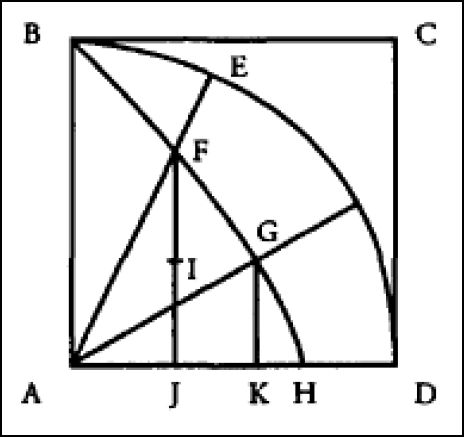). Let ABCD be a square, ABED the quadrant of a circle with radius AB. Let AB make a uniform sweeping motion through the quadrant in the same time in which BC falls uniformly to coincide with AD. The quadratix is the curve BFGH described by the intersection of the two moving straight lines. The quadratix enables one to divide an angle in any given ratio, since it is easy to prove that if the given ratio is IJ:FJ and GK is made equal and parallel to IJ, then angle GAD:angle FAD::IJ:FJ. The quadrature of the circle is more difficult. One first establishes the length of circumference of the circle by showing that: arc BED:AB :: AB:AH56 and then uses a result, associated with Archimedes57 and proved by the method of exhaustion: A circle has the same area as a right triangle with one leg equal to its radius and the other equal to its circumference. Hippias of Elis is known to us from Plato’s dialogues, where he is represented as an intellectual jack-of-all trades, who has a prodigious memory, performs numerical calculations at a speed which amazes his audience, and teaches the ‘quadrivium’, calculation (logismos), astronomy, geometry and music (Protagoras 318e). In the Theaetetus (145a) Plato represents Theatetus’ instructor Theodoras, the remaining fifth-century mathematician mentioned in Proclus’ history, as knowledgeable in the subjects of the quadrivium. Unfortunately, we know no more about Theodoras’ accomplishments than we are told in the Theaetetus; that is to say, the only thing about which we can be reasonably certain is that Theodoras taught something about incommensurability. For my purposes the important point is that Hippias and Theodoras show us that already in the fifth century the core of Plato’s scientific curriculum was being taught in Greece.58 In this chapter I have not discussed astronomy, but I hope I have made clear that if the other mathematical sciences of the curriculum had reached a high level of development by Plato’s time, the groundwork for that achievement had been firmly laid by the end of the fifth century. NOTES 1 Dates are BCE (before Common Era) unless there is an indication to the contrary. For scepticism about the Eudemian provenance of this history see Lan [8.76]. 2 Materials on the people other than Eudoxus and Archytas, who are mentioned by Proclus in the second part of his history, are collected in Lasserre [8.65]. 3 See Mueller [8.38], 161–2, 192–4 and, for a historically oriented discussion of the avoidance of proportion in Books I–IV, Artmann [8.94]. 4 I put ‘rational’ and ‘irrational’ in quotation marks to make clear that the irrationality defined in Book X (definitions 3 and 4) is importantly different from the standard modern conception. Given an arbitrary straight line r taken as ‘rational’ (rhētos), Euclid calls a straight line x ‘rational’ if and only if x and r are commensurable ‘in square’ (dunamei), i.e. if and only if the square with x as side is commensurable with the square with r as side. And a rectilineal area is called ‘rational’ or ‘irrational’ depending on whether or not the side of a square equal to the area is ‘rational’. If we think of r as of length 1, then the straight line corresponding tois ‘rational’ because the square on it is twice as large as the square on r. 5 For a discussion of Democritus as a mathematician see Heath [8.7] 1:176–81. 6 For discussion of Eudoxus’ equally impressive achievements in geometrical astronomy see, e.g. Dicks [8.16], 151–89 or Neugebauer [8.18], 675–89. 7 The dodecahedron is very complicated mathematically, but it occurs naturally as a crystal, and there are quite early fabricated versions of it. Neither of these two things is true of the complicated icosahedron. 8 I here omit the argument establishing the correlation between these means and the irrationals which underlies Pappus’ description of Theaetetus’s accomplishment. 9 Euclid also describes a straight line as dunamenos an area when it is equal to the side of a square equal to the area. For this use cf. Plato, Theaetetus I48b2. 10 This is obviously the case for the arithmetic mean. A proof in Greek style for the harmonic mean is elaborate; the core idea is that the harmonic mean between integers m and n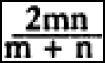. 11 See section 1 above. Book XIII only makes use of apotomes and lines related to them, not binomials. But the symmetry between binomials and apotomes would seem to provide a satisfactory explanation of the development of a theory of both. 12 Van der Waerden adopts an extreme version of this position. For him Book XIII itself was written by Theaetetus and incorporated in the Elements without revision. Book X, which was also written by Theaetetus, was changed in its’ very early parts for reasons which we will discuss in the next section, but the body of the book, ‘which is concerned with the 13 kinds of ‘irrational’ lines, was left practically unchanged by Euclid, except that he and his followers added a number of less important propositions and remarks, intended to clarify the very difficult subject’ (van der Waerden [8.13], 179). 13 In this case Euclid’s argument requires the ‘axiom of Archimedes’ (see section 1 above). 14 See Mueller [8.38] 58–72 15 Namely, x:(m-x)::y:(m-y). Cf. Heath [8.32] 3:25 and 2:126–9. 16 The idea of a pre-Eudoxian anthuphairetic conception of proportionality is most fully developed by Fowler [8.68]. 17 The same kind of view is ascribed to ‘those around Archytas and Eudoxus’ by Theon of Smyrna ([8.92], 61.11–17, DK 47 A 19a). 18 ‘Movements which are thicker produce higher notes, thinner ones lower’ ([8.30] 8: 158.8–9). 19 There is a quite full discussion in Barker [8.14], 190–208. 20 In IV.2 ([8.28], 303.19–304.6) Boethius reproduces the proof from the Sectio. 21 For discussion see Barker [8.14], 56–75. To explain the difference I first remark that it is customary to give a scale for two octaves, each divided into two fourths or tetrachords separated by a tone; each tetrachord is divided in the same way into three intervals. The standard diatonic tuning is represented by: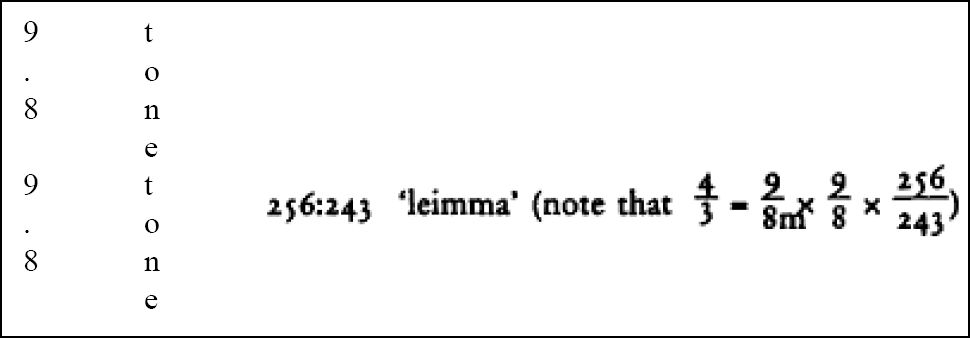whereas Archytas’ is: 9:8 8:7 28:27. 22 One could say much the same thing about the question of the basic attitude underlying Plato’s attitude toward mathematical science. Much that he says suggests to us a quite scientific outlook, but passages like the division of the world soul in the Timaeus (35a–36b) or the description of the marriage number in the Republic (546b-c) make it difficult to feel confident about his general stance or about the many passages which are vague enough to sustain both a scientific and a mystifying reading. 23 For a discussion of Archytas’ construction of a cube twice the size of a given one, which is a tour de force of the spatial imagination, see, e.g., Heath [8.7] 1: 246–9. 24 See Heath [8.7] 1:128–37. 25 See, e.g., van der Waerden [8.13], 35–6. Cf. Neugebauer’s remark ([8.10], 91) about astronomy: ‘Ancient science was the product of a very few men; and these few happened not to be Egyptians.’ 26 See Burkert [8.79], 380–2. For a defense of Heraclides of Pontus as Porphyry’s source see During [8.78], 154–7. 27 Burkert ([8.79] 378–9), wishing to stress non-Pythagorean interest in music theory, fastens on a corrupt text (Theon of Smyrna [8.92], 59.4–21, DK 18 A 13) in which a physically impossible experiment involving the striking of vessels filled to various heights with liquid, may be ascribed to Lasus of Hermione, a person from the last half of the sixth century, who, according to the Suda ([8.87] 3:236.23–7), was the first to write a book (logos) on music, to introduce dithyrambs into competition, and to introduce eristic arguments (!) 28 See Burkert [8.79], 192–207. 29 Philolaus’ two accounts of the comma are equivalent since: tone−two dieses=(tone −diesis)−diesis=apotomediesis. 30 On the tetraktus see Delatte [8.80], 249–68. 31 I here ignore difficulties involved in treating 1 as a number. For Nicomachus ([8.55], II.8.3) 1 is ‘potentially’ a triangular number. 32 On Euclid’s definition of ‘gnomon’ (Elements II, def. 2) and the origin of the word itself, see Heath, [8.32] 1:370–2. 33 Burkert ([8.79], 433–4) accepts the figurate number material as early and admits that ‘even a game may be regarded legitimately as a kind of mathematics’. But he insists on the deductive character of even Thales’ geometry, in the context of which ‘Pythagorean arithmetic is an intrusive quasi-primitive element’. 34 Even contemporary defenders of the idea of an early Pythagorean mathematics are usually willing to concede that attributions of scientific achievements to Pythagoras are always subject to question and will settle for an attribution to the ‘early Pythagoreans’, a somewhat vague locution which I take to refer to the period before 450. In this essay I stress ancient attributions to Pythagoras because they offer the greatest challenge to sceptics. I am, however, only interested in early Pythagorean science, not in the science of Pythagoras. 35 For the sources see Heath [8.7] 1:144–5. 36 [8.74], 428.10–21. In the continuation Proclus attributes a parallel method to Plato. 37 For example,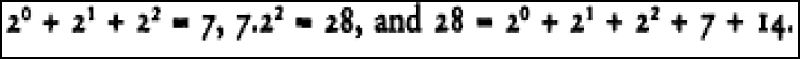. 38 Burkert also objects that Becker’s proof of IX.36 ‘requires an abundant use of modern algebraic notation’. Here I think he points to a problem which cannot be avoided in writing out an argument which turns on perceived spatial relations. The argument I have given uses the fact there are no infinitely descending sequences of integers, which is a form of what we know as the principle of mathematical induction, but which is immediately obvious from the figurate representation of numbers. On the other hand, Burkert is right to question whether the notion of perfection involved in IX.36 could have coexisted with the notion of perfection involved in calling 10, the sum of 1, 2, 3 and 4, perfect. But such a consideration does not seem to me decisive. 39 I signal, but do not discuss, another passage ([8.74], 379.2–5) in which Proclus says that Eudemus ascribed to the Pythagoreans the discovery and proof of the proposition that the sum of the angles of a triangle is equal to two right angles (Elements I.32). Burkert ([8.79], 451, n.21) apparently takes these to be ‘late’ Pythagoreans. 40 Being able to carry out VI.28 depends on a condition on b which I here ignore. 41 See, e.g., Tannery [8.107] and ch. 1 of Zeuthen [8.111]. For criticism of the algebraic interpretation with other references see Unguru [8.108]. 42 In I.45 Euclid generalizes I.44 by making b an arbitrary rectilineal figure. In [8.32] Heath gives the algebraic interpretation of I.44–5 on p. 374 of vol. 1 and that of VI. 28–9 on pp. 258–67 of vol. 2. He gives a similar and even more elaborate account of Book X on pp. 4–10 of vol. 3. For a purely geometric reading of Book X see Taisbak [8.39]. 43 See, e.g., van der Waerden [8.13], 124. 44 Cf. Neugebauer [8.10], 146–52, Burkert [8.79], 454, neither of whom share van der Waerden’s enthusiasm for the Pythagoreans. 45 But possibly made earlier by Hippasus of Metapontum as early as c.450. 46 Von Fritz [8.46], 403 refers to this technique as ‘an old one, known by craftsmen as a rule of thumb many centuries before the beginning of Greek philosophy and science’. However, he gives no evidence for this characterization. 47 As an example of this view see Raven [8.105], and for criticism the review by Vlastos (Gnomon 25 (1953): 29–35). 48 For one attempt see Hasse and Scholz [8.100], and for criticism van der Waerden [8.109]. 49 The view that Parmenides’ argumentation was the source of Greek mathematical rigour was put forward most fully in Szabó [8.100]. It is endorsed by Burkert [8. 79], 424–6. For criticism see Knorr [8.102] and the review of Szabó by Bowen (Historia Mathematical 11 (1982); 335–45). 50 See Heath [8.7] 1:172–4. 51 For discussion see Heath [8.7] 1:183–200, 220–5 and Mueller [8.103]. 52 Compare the different analyses of Rudio [8.85] and Heath [8.7] 1:183–91. 53 There are quite full discussions in Heath [8.7] 1:191–200 and Bulmer-Thomas [8. 49]. 54 For a discussion of Oinopides’ work in astronomy see Bulmer-Thomas [8.59]. 55 For the relevant texts see Heath [8.7] 1:225–6, who, however, reaches a different conclusion about Hippias and quadrature. 56 Of course, since H results when AB and BC coincide, H can only be determined as the limit of a sequence of points produced before they coincide. 57 Measurement of a Circle, prop. 1. 58 At the beginning of the fragment (DK 47 B 1) from On Mathematics referred to at the beginning of section 6 part one, Archytas also mentions the four mathematical sciences as brothers. Burkert ([8.79], 380, n. 46) questions the authenticity of the remarks, but one part of his objection rests on the text printed in DK, which combines a passage in Porphyry in which astronomy is described but not named with a passage in Nicomachus in which astronomy is called spherics, but is not described. BIBLIOGRAPHY General Works 8.1 Becker, O. Grundlagen der Mathematik in geschichtlicher Entwicklung, Freiburg and Munich, Verlag Karl Alber, 1954. 8.2 ——Das mathematische Denken der Antike, Göttingen, Vandenhoeck und Ruprecht, 1957. 8.3 ——(ed.) Zur Geschichte der griechischen Mathematik, Darmstadt, Wissenschaftliche Buchgesellschaft, 1965. 8.4 Bowen, A.C. (ed.) Science and Philosophy in Classical Greece, New York and London, Garland Publishing, 1991. 8.5 DK [2.2]. 8.6 Gillespie, C.C. (ed.) Dictionary of Scientific Biography, 16 vols, New York, Charles Scribner’s Sons, 1970–90. Cited as DSB. 8.7 Heath [2.34]. 8.8 Knorr, W.R. The Ancient Tradition of Geometric Problems Boston, Basel and Stuttgart, Birkhäuser, 1986. 8.9 Mueller, I. (ed.) . Essays on Greek Mathematics and its Later Development (Apeiron 14.4), South Edmonton, Academic Printing and Publishing, 1991. 8.10 Neugebauer, O. The Exact Sciences in Antiquity, 2nd edn. Providence, Brown University Press, 1957. 8.11 Sprague [7.4]. 8.12 Thomas, I. (ed. and trans.) Selections Illustrating the History of Greek Mathematics, 2 vols, Cambridge, Mass. Harvard University Press, 1939. 8.13 van der Waerden, B.L. Science Awakening, (trans. Arnold Dresden), New York, John Wiley and Sons, 1963. Music Theory 8.14 Barker, A. (ed.) Greek Musical Writings, vol. 2, Cambridge, Cambridge University Press, 1989. 8.15 ——‘Three approaches to canonic division’, in Mueller [8.9], pp. 49–83. Astronomy 8.16 Dicks, [2.27]. 8.17 Heath [2.33]. 8.18 Neugebauer, O. A History of Ancient Mathematical Astronomy, 3 vols, New York, Heidelberg, Berlin, Springer-Verlag, 1975. 8.19 van der Waerden, B.L. Die Astronomie der Griechen: Eine Einführung, Darmstadt, Wissenschaftliche Buchgesellschaft, 1988. Ancient Authors Cited For each author works are cited in the following order: (1) original text, (2) translation, (3) secondary works in alphabetical order. Alexander of Aphrodisias 8.20 Wallies, M. (ed.) Alexandri Aphrodisiensis in Aristotelis Topicorum Libros Octo Commentaria (Commentaria in Aristotelem Graeca II.2), Berlin, George Reimer, 1891. Antiphon (DK 80) 8.21 Morrison, J.S. (trans.) ‘Antiphon’, in Sprague [7.4], pp. 106–240. English translation. Archimedes 8.22 Heiberg, J.L. (ed.) Archimedis Opera Omnia cum Commentariis Eutocii, 2nd edn, vols 1 and 2, Leipzig, B.G.Teubner, 1910, 1913. 8.23 Heath, T.L. The Works of Archimedes, 2nd edn, Cambridge, Cambridge University Press, 1912. Aristotle 8.24 Bekker, I. (ed.) Aristoteles Graece, Berlin, George Reimer, 1831. 8.25 Barnes, J. (ed.) The Complete Works of Aristotle, Princeton, NJ, Princeton University Press, 1984. English translation. 8.26 Heath, T. Mathematics in Aristotle, Oxford, Clarendon Press, 1949. Archytas (DK 47) 8.27 Bowen, A.C. ‘The foundation of early Pythagorean harmonic science: Archytas, fragment 1’, Ancient Philosophy 2 (1982): 79–94. Boethius 8.28 Friedlein, G. (ed.) Boetii De Institutions Arithmetica. De Institutions Musica, Leipzig, B.G.Teubner, 1867. 8.29 Bower, C.M. (trans.) Anicius Manlius Severinus Boethius, Fundamentals of Music, New Haven and London, Yale University Press, 1989. English translation. Euclid 8.30 Heiberg, J.L. and Menge, H. (eds) Euclidis Opera Omnia, 9 vols, Leipzig, B.G.Teubner, 1883–1916. (Vols 1–4 contain the Elements, and vol. 5 the scholia on the Elements; the Sectio Canonis is printed in vol. 8.) 8.31 Stamatis, E.S. (ed.) Euclidis Elementa, 4 vols, Leipzig, B.G.Teubner, 1969–73 (a second edition of the first four volumes of Heiberg and Menge [8.30]). 8.32 Heath, T.L. The Thirteen Books of Euclid’s Elements, 2nd edn, 3 vols, Cambridge, Cambridge University Press, 1926. The Sectio Canonis is translated in Barker [8.14], pp. 190–208. English translation. 8.33 Artmann, B., ‘Euclid’s Elements and its prehistory’, in Mueller [8.9], 1–47. 8.34 Barker, A., ‘Methods and aims in the Euclidean “Sectio Canonis”’, Journal of Hellenic Studies 101 (1981): 1–16. 8.35 Bowen, A.C. ‘Euclid’s Sectio Canonis and the history of Pythagoreanism’, in Bowen [8.4], pp. 164–87. 8.36 Bulmer-Thomas, I. ‘Euclid’, in DSB [8.6], 4:414–37. 8.37 Knorr, W.R. The Evolution of the Euclidean Elements, Dordrecht and Boston, D.Reidel, 1975. 8.38 Mueller, I. Philosophy of Mathematics and Deductive Structure in Euclid’s Elements, Cambridge, Mass, MIT Press, 1981. 8.39 Taisbak, C.M. Coloured Quadrangles: A Guide to the Tenth Book of Euclid’s Elements, Copenhagen, Museum Tusculanum Press, 1982. Eudemus 8.40 Wehrli, F. (ed.) Eudemos von Rhodos (=Die Schule des Aristoteles 8), Basel, Benno Schwabe, 1955. Eudoxus 8.41 Lasserre, F. Die Fragmente des Eudoxos von Knidos, Berlin, Walter de Gruyter, 1966. 8.42 Huxley, G. ‘Studies in the Greek astronomers I. Eudoxian topics’, Greek, Roman, and Byzantine Studies 4 (1963): 83–96. 8.43 Stein, H. ‘Eudoxus and Dedekind, on the ancient Greek theory of ratios and its relation to modern mathematics’, Synthese 84 (1990): 163–211. Eutocius 8.44 Heiberg, J.L. (ed.) Archimedis Opera Omnia cum Commentariis Eutocii, 2nd edn, vol. 3, Leipzig, B.G.Teubner, 1915. Heron 8.45 Schmidt, W. Nix, L., Schöne, H. and Heiberg, J.L. (eds and trans) Heronis Alexandrini Opera quae Supersunt Omnia, 5 vols, Leipzig, B.G.Teubner, 1899– 1914. Hippasus of Metapontum (DK 18) 8.46 von Fritz, K. ‘The discovery of incommensurability by Hippasus of Metapontum’, Annals of Mathematics 46 (1945), repr. in and cited after Allen and Furley [2.15] I: 282–412. Hippias of Elis (DK 86) 8.48 Gallop, D. (trans.) ‘Hippias’, in Sprague [7.47], pp. 94–105. English translation. 8.47 Bulmer-Thomas, I. ‘Hippias of Elis’, in DSB [8.6] 6:405–10. Hippocrates of Chios (DK 42) 8.49 Bulmer-Thomas, I. ‘Hippocrates of Chios’, in DSB [8.6] 6:410–18. 8.50 Lloyd, G. ‘The alleged fallacy of Hippocrates of Chios’, Apeiron 20 (1987): 103–28. Iamblichus 8.51 Deubner, L. and Klein, U. (eds) lamblichi de Vita Pythagorica Liber, Stuttgart, B.G.Teubner, 1975. 8.52 Guthrie, K.S. (ed., and trans.) The Pythagorean Sourcebook and Library, Grand Rapids, Mich., Phanes Press, 1987, pp. 57–122. English translation. 8.53 Festa, N. and Klein, U. (eds) lamblichi de Communi Mathematica Scientia Liber, Stuttgart, B.G.Teubner, 1975. 8.54 Pistelli, H. and Klein, U. (eds) lamblichi in Nicomachi Arithmeticam Introductionem Liber, Stuttgart, B.G.Teubner, 1975. Nicomachus 8.55 Hoche, R. (ed.) Nicomachi Geraseni Pythagorei Introductions Arithmeticae Libri II, Leipzig, B.G.Teubner, 1866. 8.56 D’Ooge, M.L. (trans.) Nicomachus of Gerasa, Introduction to Arithmetic, New York, The Macmillan Co., 1926. English translation. 8.57 von Jan, K. (ed.) in von Jan, K. (ed.) Musici Scriptores Graeci, Leipzig, B.G.Teubner, 1895, 237–65. 8.58 Barker [8.14], 245–69. English translation. Oinopides (DK 41) 8.59 Bulmer-Thomas, I. ‘Oenopides’, in DSB [8.6] 10:179–182. Pappus 8.60 Thomson, W. (ed. and trans.) The Commentary of Pappus on Book X of Euclid’s Elements (Harvard Semitic Series 8), Cambridge, Mass. Harvard University Press, 1930. Philolaus (DK 44) 8.61 Huffman, [4.78]. Plato and the Academy 8.62 Burnet, J. (ed.) Platonis Opera, 5 vols, Oxford, Clarendon Press, 1900–7. 8.63 Hamilton, E. and Cairns, H. (eds) The Collected Dialogues of Plato, Princeton, NJ, Princeton University Press, 1971. English translation. 8.64 Greene, W.C. (ed.) Scholia Platonica (American Philological Association Monographs 8), Haverford, Pa., American Philological Society, 1938. 8.65 Lasserre, F. (ed.) De Léodamas de Thasos à Philipe d’Oponte, Naples, Bibliopolis, 1987. 8.66 Anton, J. (ed.) Science and the Sciences in Plato, Albany, NY, Eidos Press, 1980. 8.67 Cherniss, H. ‘Plato as mathematician’, Review of Metaphysics 4 (1951): 395–405, repr. in Selected Papers, ed. L.Tarán, Leiden, E.J.Brill, 1977, pp. 222–52. 8.68 Fowler, D.H. The Mathematics of Plato’s Academy, Oxford, Oxford University Press; paperback edn with addenda, 1991. 8.69 Frajese, A. (ed.) Platone e la matematica nel mondo antico, Rome, Editrice Studium, 1963. 8.70 Mueller, I. ‘Mathematics and education, notes on the Platonist program’, in Mueller [8.9], pp. 85–104. 8.71 ——‘Mathematical method and philosophical truth’, in R.Kraut (ed.) The Cambridge Companion to Plato, Cambridge, Cambridge University Press, 1992, pp. 170–99. Plutarch 8.72 Plutarch’s Moralia, 15 vols, Cambridge, Mass. Harvard University Press, 1927–69. Porphyry 8.73 Düring, I. (ed.) Porphyrios Kommentar zur Harmonielehre des Ptolemaios, Göteborg, Elanders Boktryckeri Aktiebolag, 1932. Proclus 8.74 Friedlein, G. (ed.) Procli Diadochi in Primum Euclidis Elementorum Librum Commentarii, Leipzig, B.G.Teubner, 1873. 8.75 Morrow, G.R. (trans.) Proclus, A Commentary on the First Book of Euclid’s Elements, Princeton, NJ, Princeton University Press, 1992. English translation. 8.76 Lan, C.E. ‘Eudemo y el “catalogo de géometras” de Proclo’, Emerita 53 (1985): 127–57. Ptolemy 8.77 During, I. (ed.) Die Harmonielehre des Klaudios Ptolemaios, Göteborg, Elanders Boktryckeri Aktiebolag, 1930. 8.78 ——(trans.) Ptolemaios und Porphyrios über die Musik, Göteborg, Elanders Boktryckeri Aktiebolag, 1934. Pythagoras and the Pythagoreans (DK 14–20, 58) 8.79 Burkert, [2.25]. 8.80 Delatte, A. Études sur la Littérature Pythagoricienne, Paris, Librairie Ancienne Honoré Champion, 1915. 8.81 von der Waerden, B.L. Die Pythagoreer, Zürich and Munich, Artemis Verlag, 1979. 8.82 Zhmud, L. ‘“All is number”?’, Phronesis 34 (1989): 270–92. 8.83 ——‘Pythagoras as mathematician’, Historia Mathematica 16 (1989): 249–68. Simplicius 8.84 Diels, H. (ed.) Simplicii in Aristotelis Physicorum Libros Quattuor Priores Commentaria (Commentaria in Aristotelem Graeca IX), Berlin, George Reimer, 1882. 8.85 Rudio, F. (ed. and trans.) Der Bericht des Simplicius über die Quadraturen des Antiphons und des Hippokrates, Leipzig, B.G.Teubner, 1907. Stobaeus, Joannes 8.86 Wachsmuth, C. and Hense, O. (eds) loannis Stobaei Anthologium, 5 vols, Berlin, Weidmann, 1884–1912. Suda 8.87 Adler, A. (ed.) Suidae Lexicon, 4 vols, Leipzig, B.G.Teubner, 1928–35. Thales (DK 11) 8.88 Dicks, D.R. ‘Thales’, Classical Quarterly NS 9 (1959): 294–309. Theaetetus (Lasserre [8.65] 3) 8.89 Bulmer-Thomas, I. ‘Theaetetus’, in DSB [8.6] 13:301–7. 8.90 Thesleff, H. ‘Theaitetos and Theodoros’, Arctos 24 (1990): 147–59. Theodorus of Cyrene 8.91 Bulmer-Thomas, I. ‘Theodorus of Cyrene’, in DSB [8.6] 13:314–19. Theon of Smyrna 8.92 Hiller, E. (ed.) Theonis Smyrnaei Philosophi Platonici Expositio Rerum Mathematicarum ad Legendum Platonem Utilium, Lepizig, B.G.Teubner, 1878. 8.93 Lawlor, R. and D. (trans.) Theon of Smyrna, Mathematics Useful for Understanding Plato, San Diego, Calif., Wizards Bookshelf, 1979. English translation. Other Secondary Sources 8.94 Artmann, B. ‘Über voreuklidische “Elemente”, deren Autor Proportionen vermied’, Archive for History of Exact Sciences 33 (1985): 292–306. 8.95 Becker, O. ‘Die Lehre von Geraden und Ungeraden im neunten Buch der euklidischen Elemente’, Quellen und Studien zur Geschichte der Mathematik, Astronomie und Physik, Abteilung B. 3 (1936): 533–53; reprinted in Becker [8.3], pp. 125–45. 8.96 ——‘Eine voreudoxische Proportionenlehre und ihre Spuren bei Aristoteles und Euklid’, Quellen und Studien zur Geschichte der Aathematik, Astronomie und Physik, Abteilung B. 2 (1933): 311–33. 8.97 von Fritz, K. ‘Die APXAI in der griechischen Mathematik’, Archiv für Begriffsgeschichte 1 (1955): 13–103; repr. in von Fritz [8.99], pp. 335–429. 8.98 ——‘Gleichheit, Kongruenz und Ähnlichkeit in der antiken Mathematik bis auf Euklid’, Archiv für Begriffsgeschichte 4 (1959): 7–81; repr. in von Fritz [8.99], 430–508. 8.99 ——Grundprobleme der Geschichte der Antiken Wissenschaft, Berlin and New York, Walter de Gruyter, 1971. 8.100 Hasse, H. and Scholz, H., Die Grundlagenkrisis der griechischen Mathematik, Charlottenburg, Pan-Verlag Kurt Metzner, 1928. 8.101 Knorr, W.R. ‘On the early history of axiomatics, the interaction of mathematics and philosophy in Greek antiquity’, in J.Hintikka, D.Gruender and E. Agazzi (eds) Theory Change, Ancient Axiomatics, and Galileo’s Methodology (Proceedings of the 1978 Pisa Conference on the History and Philosophy of Science, vol. 1), Dordrecht, D.Reidel, 1981. 8.102 KRS [1.6]. 8.103 Mueller, I. ‘Aristotle and the quadrature of the circle’, in N.Kretzmann (ed.) Infinity and Continuity in Ancient and Medieval Thought, Ithaca, NY, Cornell University Press, 1982, pp. 146–64. 8.104 ——‘On the notion of a mathematical starting-point in Plato, Aristotle, and Euclid’, in Bowen [8.4], pp. 59–97. 8.105 Raven, J.E. Pythagoreans and Eleatics, Cambridge, Cambridge University Press, 1948. 8.106 Szabó, A. The Beginnings of Greek Mathematics, trans. A.M.Ungar, Dordrecht, D.Reidel, 1978. 8.107 Tannery, P. ‘De la solution géométrique des problèmes du second degré avant Euclide’, Mémoires de la Société des Sciences Physiques et Naturelles de Bordeaux, 2e série, 4 (1882): 395–416; repr. in P.Tannery Mémoires Scientifiques, 17 vols, Toulouse, Edouard Privat and Paris, Gauthier-Villars, 1912–50, vol. 1, pp. 254–80. 8.108 Unguru, S. ‘History of ancient mathematics: some reflections on the state of the art’, Isis 70 (1979): 555–65. 8.109 von der Waerden, B.L. ‘Zenon und die Grundlagenkrise der griechischen Mathematik’, Mathematische Annalen 117 (1940): 141–61. 8.110 Waterhouse, W.C. ‘The discovery of the regular solids’, Archive for History of Exact Sciences 9 (1972–3): 212–21. 8.111 Zeuthen, H.G. Die Lehre von den Kegelschnitten im Altertum, trans. R.V. Fischer- Benzon, Copenhagen, Verlag von Andr. Fred. Høst & Søhn, 1886.

Routledge History of Philosophy. . 2005.

### Look at other dictionaries:

• Proclus — See Exact sciences (The) in Hellenistic times: texts and issues, see Greek arithmetic, geometry and harmonics: Thales to Plato, see …   History of philosophy